Here are some updates (mini blog) on The graph spectrum of barycentric refinements and Universality for Barycentric subdivision and Barycentric characteristic numbers.
• May 27, 2017: : here are some slides authored in the fall 2015 and spring 2016. There is some addition as for the connection Laplacian, the Barycentric limit shows a mass gap. Also on the quantum calculus blog are some new doodles from how to get a grip on the quantum plane. I decided to put the slightly updated slides on the Barycentric limit story on youtube rather having it rotting and lost on the hard drive:
• October 21, 2015: Here are explicit "coordinate transformations" in the case d=6 to go from Barycentric invariants, the eigenvectors of the 7x7 matrix AT
```  u2 = {0, -7898, 11847, -14360, 16155, -17528, 18627}
u4 = {0, 0, 0, -58, 145, -245, 350}
u6 = {0,0,0,0,0,-2,7}
```
to the classical Dehn-Sommerville-Klee invariants given by Binomial coefficients
```  v1 = {0,-2,3,-6,10,-15,21}
v2 = {0,0,0,-2,5,-15,35}
v3 = {0,0,0,0,0,-2,7}
```
The relation is
```  29 u2 + 4667 u4 + 33044 u6 = 114521 v1
u4 +    95 u6 =     29 v2
u6 =        v3
```
How does the explicit integer coordinate transformation look like in general? Here is an other example: the basis change matrix which maps the Dehn-Sommerville numbers to the Barycentric numbers. I don't see the formula for the matrix entries yet. We know the Dehn-Sommerville numbers explicitly as we do know the eigenvectors of the Barycentric matrix (the Barycentric characteristic numbers). So, in principle, we could get "really messy" formulas for the basis change matrix.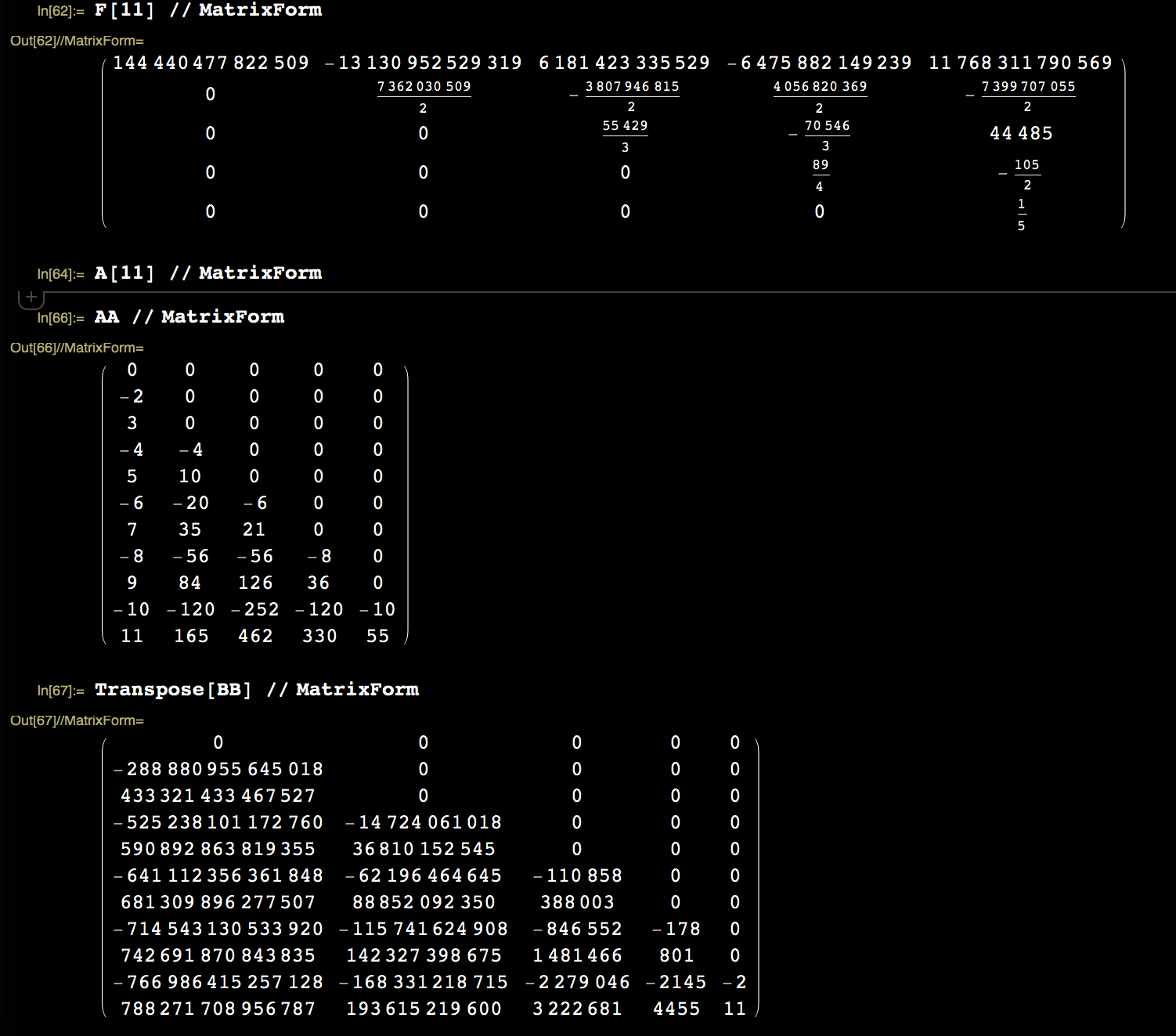• October 15, 2015: The language of graph theory is less familiar in the context of Barycentric refinement. While the concept of simplicial complexes is more general, it is actually enough to look at graphs. There are two point of views: one is to see a simplicial complex as an additional structure on a set like a topological, measure an order or algebraic structure: a simplicial complex is simply a subset of the set of subsets which is closed under forming subsets. There is an other point of view, which is taken in geometric probability theory: given a simplicial complex, one can look at the lattice generated by all simplicial sub complexes and define so valuations. If the set of sub complexes form the vertex set of a graph, and if we form the edge set as the set of pairs where one is contained in the other, then this defines a graph which contains all the essential information about the complex. If the simplicial complex was the Whitney of a graph, then the new graph is just the Barycentric refinement. In any case, we see that it does not matter whether we start with a simplicial complex or a graph when doing refinements. We always get already after one step to complexes which can be described by graphs. So, when looking at Barycentric refinements, and especially when iterating them, we have no reason to carry around the language of simplicial complexes. Just start with a graph and define from it a new graph. This is not only simpler, it also removes any possible confusion as Barycentric refinement is usually considered in an Euclidean setting or for structures embedded in an Euclidean space.
• October 14, 2015: A rehearsal for a seminar. .
• October 13, 2015: Start with the spectrum {l(1),..,l(n)} of a graph, then there is no reason why the logarithmic energy sum_(i not equal to j) -log|l(i)-l(j)| should be minimal when restricted to an interval [0,max( spectrum(L))]. What happens in the one dimensional case is that under refinement, the spectrum gets closer and closer to the minimal logarithmic point set energy. This follows from the spectral measure convergence in one dimensions. To describe such a situation in the 2 or higher dimensional case is more difficult as we don't know the nature of the support of the measure in the limit. But its reasonable to think that the logarithmic energy of the spectral measures converges to the minimum once we know the gaps in the spectrum. Unfortunately we have not even shown the existence of a single gap in the spectrum.
• October 12, 2015:
 Is the limiting group indeed just the product of two dyadic groups of integers? The presentation of the limiting group will no more be finite but isomorphic as a group. Here is a simple example: the group Z4 can be generated by one generator or by two. The group stays the same but the Cayley graph changes and becomes triangular. The limiting group of the Barycentric refinement has a countable set of generators and many relations. If we look at the limiting random ergodic operator, then any representation as a matrix in some basis has infinitely many entries in most rows and columns. This is obvious also from the fact that the limiting Laplacian is an unbounded operator, unlike in one dimensions, where it is a bounded Jacobi matrix. An example of an infinitely generated presented group is Z with generators ak(x) = x+2^k which has the property that the generators satisfy the relations ak^2 = a k+1. The Cayley graph has now infinite vertex degree at each vertex and the corresponding Laplacian is unbounded. The original Laplacian of Z is the free Jacobi matrix, the limiting Barycentric operator in one dimensions. There are two ways to see more structure: one is ergodic theory as discussed below (fixed point of a 2:1 integral extension map among dynamical systems), the other is potential theory as the equilibrium measure on the spectrum [0,4] is the arc sin distribution which is invariant under the quadratic map having the spectrum as Julia set. One could experimentally check whether the density of states in the two dimensional case is the equilibrium measure on the spectrum as a set, minimizing the logarithmic energy, the measure which leads to logarithmic capacity.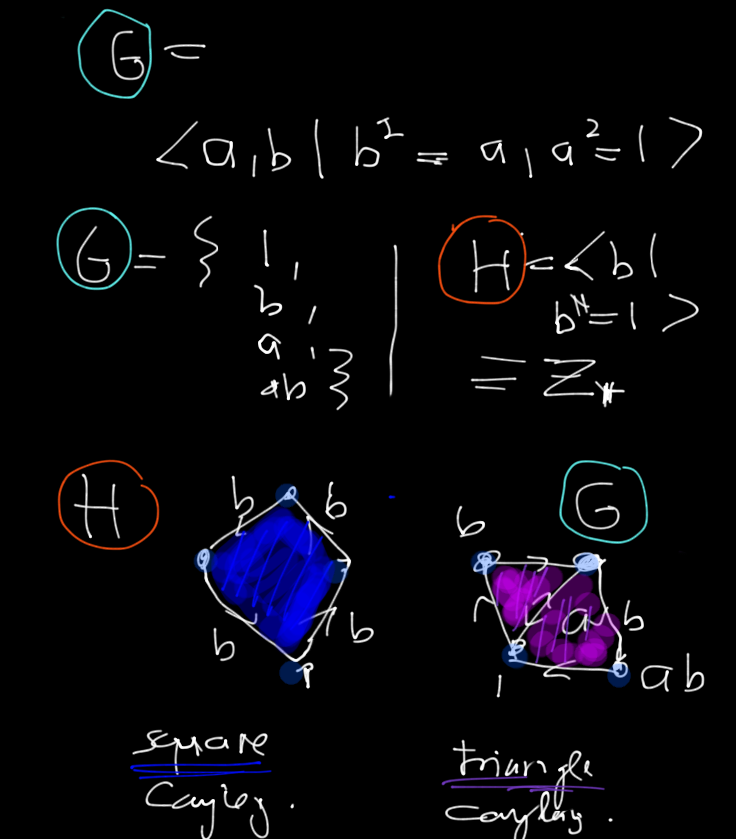• October 11, 2015:

 The Z2 dynamical system renormalization defines a refinement of the grid, but we need other finitely presented groups to model the Barycentric refinement. For d=2, we need 6 generators already after one step. On each triangle, we have to renormalize (S,T,R) -> (S',T',R',U',V',W'). We do that with four intervals. The first one belongs to the vertices, the two next ones to edges and the final one to triangles. First double S and T, form R. Now define U as half of TS. Then define V,W. Now we have 6 transformations on the interval. An orbit of the so obtained finitely presented group defines the Barycentric subdivision. In the next refinement, we have to renormalize in each triangle and get a group with 12 generators. The generators can all be obtained from S,T and their refinements but we need the new generators to define the graph structure and so the Laplacian at each stage. The frame work of ergodic theory has an advantage about inverse limit constructions as there is a natural completeness built in already: taking a sequence of group actions, the limiting object already part of the setup.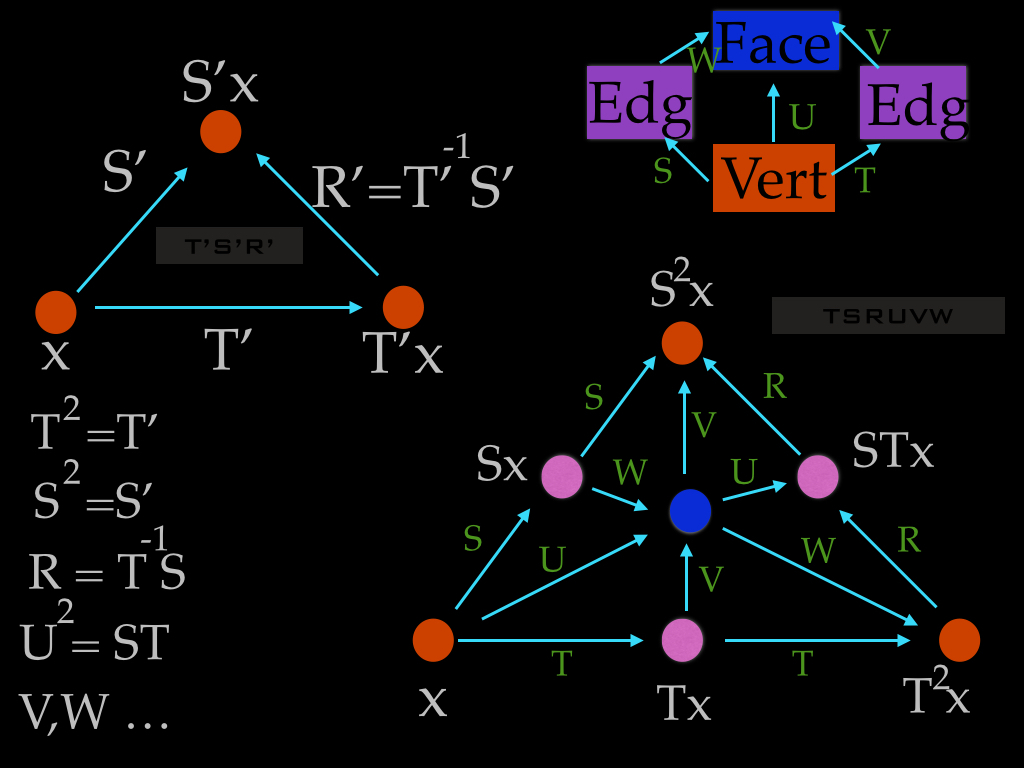October 10, 2015: The Zd action as the Barycentric picture is simplex based and the Zd action cube based. How do we bring the Barycentric picture into the dynamical picture? We might have to take more serious an other meaning of "Barycentric". It is the concept of Barycentric coordinates. Similarly as projective geometry uses an additional redundant variable to allow dealing with infinity (the stereographic projection from a translated plane to a sphere allows to work with a projective compact space instead), Barycentric coordinates add a third coordinate z to describe a point in a triangle. The picture is to look at the triangle cut out from the plane x+y+z=1 in the first quadrant. The coordinates (x,y,z) of a point on this triangle now always satisfy x+y+z=1 and are more symmetric. Why? The center of the triangle is now nicely (1/3,1/3,1/3) and the centers of the triangle vertices have the coordinates (1,0,0),(0,1,0),(0,0,1) and the edges have the coordinates (1/2,1/2,0),(0,1/2,1/2),(1/2,0,1/2). This is much nicer than what happens with 2 variables only, where (0,0,0,(1,0,0),(1/2,31/3/2,0) are the vertices and the edge and triangle centers are similarly asymmetric. We will have to incorporate this into the dynamical picture. This will make it necessary to refine the lattice action by additional generators. The renormalization map will be a map on finitely presented groups acting on the probability space. [ Digression: Barycentric coordinates adds both redundancy and symmetry. Similarly as a hash code. A point (0.5,0,0.6) for example can not be right since the coordinates do not add up to zero. Barycentric coordinates form a simple error correcting code. Going into higher dimensions is not only used in relativity, computer vision which is a particular application of projective geometry, it is also a well known method to construct quasi crystals: take a lattice in d+1 dimensions, chose a random hyperplane, then look at the positions of the projections of the nearest lattice points to this plane. This lattice is no more periodic for most hyperplanes but an almost periodic point configuration. I proved once that among packings obtained like that, the densest packings are periodic: paper [PDF]. It actually had been a bit of a disappointment for me, as I had hoped that the computer would find record packing densities in higher dimensions, where the old Kepler packing problem is not yet solved. My interest at that time in packings came of course because of the at that time ongoing controversy about proofs of the Kepler conjecture, which now seems settled but is proven only (similarly like the 4 color problem) using computer assistance. ]
Lets look at the triangle and label one of the vertices with x Now look at the orbit the integral extension dynamical system in which the original vertices have the labels x,T^2(x),S^2(x). Now introduce a new map T(x)=T(x)S-1x. The orbit of the Z3 action generated by S,T,R is now related with a triangulation of the plane. Given a finitely presented group A acting on a probability space, equip the orbit of a point x with a graph structure by connecting two vertices x,y with an edge if there exists (n,m,k) with max(|n|,|m|,|k|)=1 such Tn Sm Uk(x)=y. Of course, if we replace the action (T,S) with a refined action (T',S') this orbit graph stays the same. This means that we will have to introduce more and more generators. Still stuck.
• October 9: Besides the translations, there is also a renormalization map which changes the dynamical structure. Given a point x, look at its orbit under the Zd action. The partition of space into 2d intervals naturally defines itinaries which is a coloring on the Zd lattice: to each vertex is attached the label of the interval telling where the point is. The renormalization defines a map on orbits: take a point x and look at its itinary under the dynamical system. It defines now a new point by looking at the orbit of the renormalized system. The itinary of the renormalized system is the new point. We could think of D2d it as a quantum d-dimensional torus as there are natural smallest translations giving us dynamically some coordinates given by sequences of numbers. The renormalization map allows us to zoom in and out. Now we have defined the space of points. What are the possible connections between points? Two points are connectable if one can translate one into the other. Can we define the circle in the dyadic plane through a valuation? What is nice about the dyadic integers is that they form a ring, are a compact space and have a Haar measure. The addition is compatible with the metric. Given the group structure, since the addition is uniquely ergodic, the metric is determined. There is no other group structure which has the property that its invariant metric is unique. An interesting question is whether there is a dihedral analogue. Start with the dihedral line and complete it with respect to the valuation. Can we define at every point exactly (d+1)! nearest translations if we define a graph structure on the orbit space? Having a graph structure on an orbit of the Zd action will also allow us to define the Laplacian: there are d! functions taking value 1 and 0 on the group (which as a Lebesgue space can be written as [0,1]) and one function taking the value -d! and 0. Given an initial point on the group (rsp a generic point on [0,1]), the limiting Laplacian will be matrix acting on l2(G^), where G^ is the dual group of G. This is analogue to how Jacobi matrices are defined in one dimension by taking two functions a,b on the circle G, taking a group translation on the circle and defining the off and diagonal matrix entries a(xn), b(xn) which are functions on the dual group G^ = Z, the integers. But again, as pointed out already, the dyadic picture is more natural in that the minimal group translation on the dyadic product of integers is unique and space is quantized. It will have quite remarkable properties like that there will be a dense set of points on that group on which there is a local orthogonal SO(d) symmetry. We can see that when we make Barycentric subdivisions and stay at some point. Under refinements, also the unit sphere gets refined (even so space gets refined also between so that it does not remain the unit sphere in the graph sense). In any case, the limiting space has remarkable similarities to our Euclidean space Rd but it is compact, has smallest translation units and a Laplacian with interesting spectral properties.

This group is equipped with a natural renormalization map which allows to "zoom in and out". This renormalization map is what was just defined on the dynamical level. The goal is to to define a random Laplacian on this group G for which the density of states is the limiting measure of the spectral central limit theorem. We need to implement the geometric Barycentric refinement within the dynamical system. It actually will be just related to inverse shift as before in one dimensions.

The Laplacian will be clear, once we have implemented a graph structure on the orbit set. This seems to be a key idea. Instead of looking at the group elements themselves, which are of course in one to one correspondence to the initial points, they have more structure. For example, while it does not makes sense to say a "point" is contained in a "point" y. It makes sense that the "orbit of x" is contained in the "orbit of y" since both are discrete sets. The orbit set V becomes a natural graph if we connect two orbits, if one is contained in the other. Let G be a finite simple graph, which is a subgraph of this graph. Now apply the inverse shift to it. This produces a new set of orbits and so a new subgraph. This is the Barycentric refinement. But: Why do we have d! neighbors for some points?
•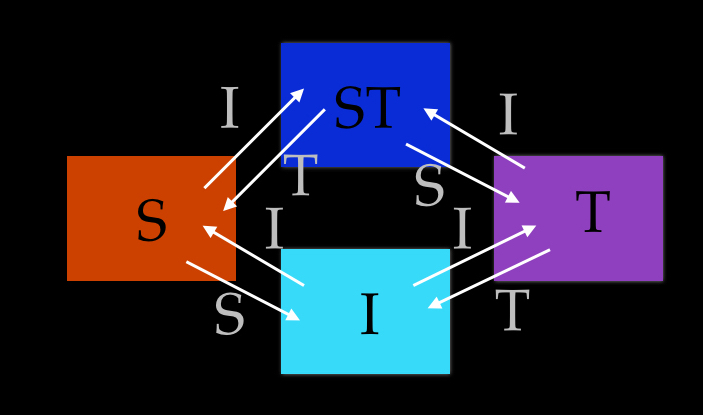October 8: the limiting group in dimension d can also be obtained from ergodic theory as the dyadic integers can be constructed from within ergodic theory. I actually defined the renormalization step as a graduate student already on the dynamical level. I just did not look at the fixed point yet then. But it is the same story as in one dimensions. As a group, the limiting space will be the group D2d, the d'th direct product of the dyadic group of integers. So, it is indeed again a profinite group as the product of profinite groups is profinite. Lets start with an arbitrary measure-preserving Zd action on ([0,1],m) where m is the Lebesgue measure on the interval [0,1]. Again, in the measure theoretical framework, taking the interval is not a loss of generality, it is similar than using separable Hilbert spaces only). Now take 2d copies of this interval and associate each interval with a subset of I={1,...,d}. This gives 2d intervals. Place the normalize Lebesgue measure on the union of the intervals. Define a new Zd action by defining Ti from an interval A to B if B = A u {i} to be the identity and by defining Ti from A to B to be the old Ti if B= A {i}. If the old dynamical system was a Zd action, the new system is again a Zd action. This multi-dimensional integral extension of Zd actions was defined in this paper [PDF]. In the same way than in 1 dimension, one can now define a uniform metric on the set of all Zd actions on [0,1]. It is given by the distance d(T,S) defined as the Lebesgue measure of all x for which one Ti(x) disagrees with Si(x). Again, this is a complete metric space and the integral extension renormalization map is a contraction, as it halfs the distance between two initial points in each step (after n steps, the maps agree on 1-2n of the interval. The Koopman operator (U_1,...,U_d) on the product Hilbert space L2(I) x ... x L2(I) has again discrete product spectrum in T1 x ...xT1. Each of the generators Ti has the dyadic complex numbers exp(i k/2n) as eigenvalues of its Koopman unitary operator. The limiting dynamical system is a uniquely ergodic Zd action on the compact topological group D2d. Each transformation generating the Zd action is almost periodic and isomorphic to the adding machine on a quotient space similarly than any translation on Rd is a translation on R when taking the quotient with the orthogonal complement of an orbit. Next, we will have to realize the Barycentric refinement inside this picture and then define the Laplacian, the almost periodic random operator which is the Barycentric limit of Laplacians on graphs. Here is an illustration from page 212 of my thesis which illustrates the convergence of the renormalization map on dynamical systems in one dimensions leading to the piecewise linear interval map called von Neumann-Kakutani system, which is the addition on the dyadic group of integers. One can see graphically the selfsimilar structure and that taking the return map onto one of the half intervals produces the same picture. This picture does not do justice to the situation in higher dimensions as that one will be much more rich.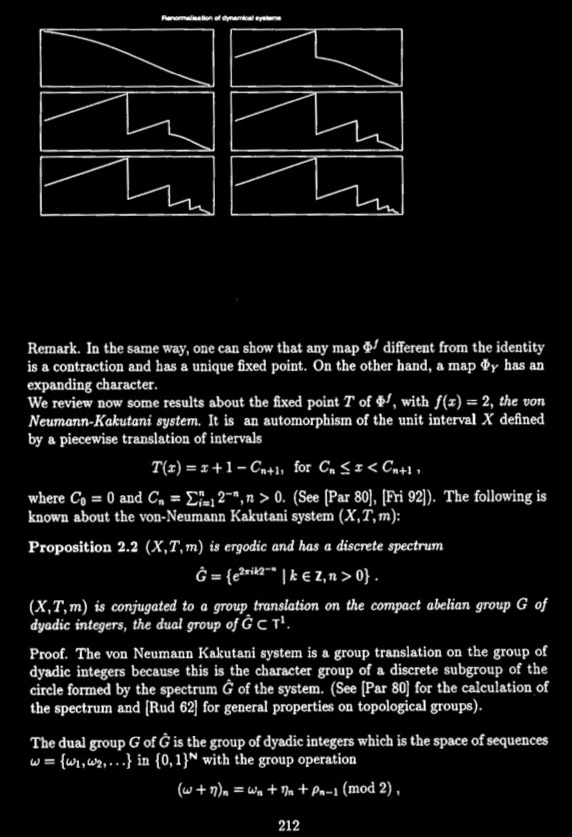• October 6, 2015: Here was my abstract to "Barycentric characteristic numbers".
"We look at functionals on graphs obtained from Barycentric subdivision. We show that for even k+d, the k-th Barycentric characteristics is zero on d-graphs, a class containing nice triangulations of compact d-manifolds. The vanishing of these numbers generalizes the fact that for such graphs, the first one, the Euler characteristic, is zero in odd dimensions. It reflects a redundancy noticed first by Klee when generalizing the Dehn-Sommerville invariants from spheres to general manifolds. It is similar to how Poincare duality renders half the Betti numbers redundant. Our proof is discrete integral geometric and uses general results for finite simple graphs or networks: after introducing curvature and index for an arbitrary valuation and stating the discrete Gauss-Bonnet and Poincare-Hopf theorems, we show that curvature is the expectation of integer divisors when integrating over a nice probability space of functions. We then express a symmetrized index at a vertex as an invariant for hypersurfaces in unit spheres, allowing to use induction. Each of the curvatures can be seen now as an average of lower dimensional sectional curvatures. The Barycentric functionals resemble so geometric functionals appearing in physics. An example is that for any triangulation of a compact 4-manifold with v vertices, e edges, f triangles, t tetrahedra, and p pentatopes, the second characteristics 22e-33f+40t-45p is zero and the associated curvature zero in the interior of a 4-manifold with boundary. The Euler characteristic v-e+f-t+p can be written in terms of the expectation of Euler characteristic of two-dimensional discrete surfaces." It was maybe foolish to submit a 2 page version without references to the ArXiv as it did not go through (this is a first for me): the message said "Our volunteer moderators determined that your article does not contain sufficient original or substantive research to merit inclusion within arXiv." They are probably right, but its kind of ironic. The work on Barycentric numbers while done in a very short time culminates for me 4 years of hard work on these themes. The proof appears to me very original even so it needed only a few sleepless nights. But it needed pulling together results developed over years. While certainly a result of modest complexity (I consider myself an amateur research mathematician by definition: one is professional is what one does most of the time), it is one of the most beautiful constructions I have done. Actually, it had been extremely tough to write in the pre-determined selfimposed 2 page limit (I wanted it to be tweetable) I had submitted the 2 pages of PDF only. The references are added now. I had felt that more references than content would look silly. Anyway, the extremely small file size and lack of references (for the record, here is the originally submitted 6917 Byte LaTeX file) must have triggered a flag at the ArXiv. I guess there is a 10K threshold beyond which somebody looks at it). I don't blame them. Some moderation is necessary and moderators are not referees. They have to make decisions in split seconds. You judge: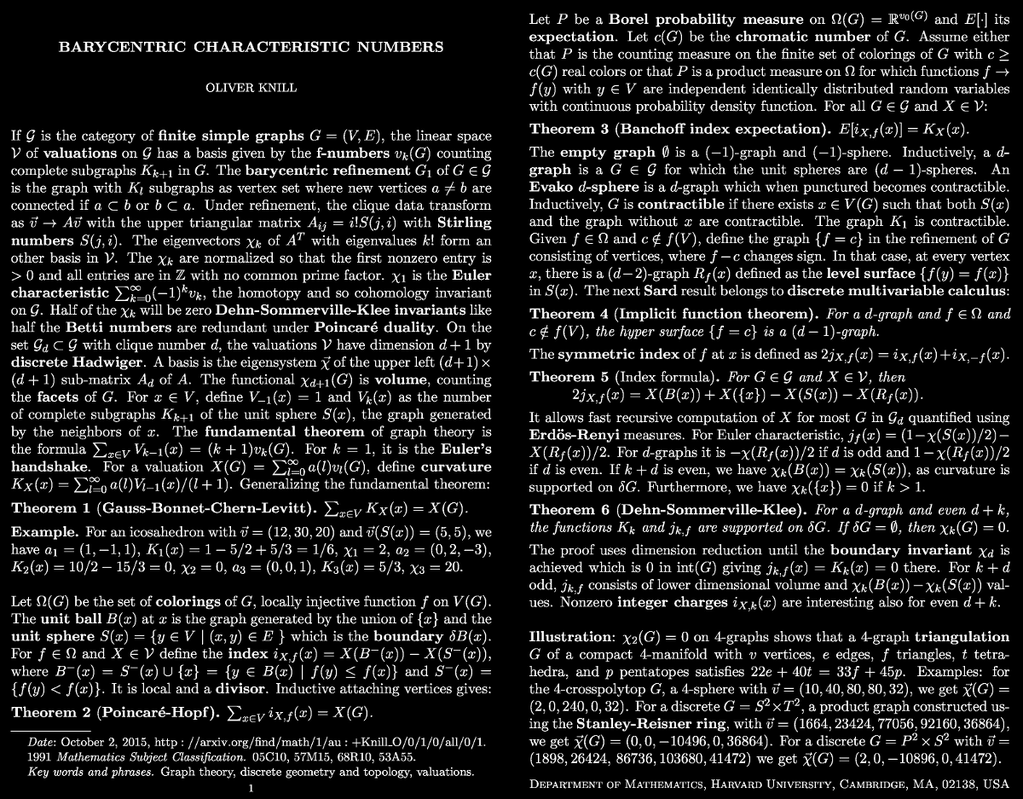Yes, the result is related to known results in other contexts but the integral theoretic proof of the vanishing of the Barycentric numbers involves a combination of nice ideas. The fact that the newly defined curvatures are concentrated on the boundary of a d-manifolds and averages of ``charges" (also not defined before for general valuations) is of great interest as it resembles what charges do in a solid. The Barycentric functionals Xk have a huge potential of being relevant in physics. They are related to symmetries and any symmetry is interesting in physics. For example: the reason why some forces needed longer to be discovered is that they average to zero. Gravity, like Euler characteristic, is obvious as it does not average to zero. For the electro magnetic forces, they needed more subtle experiments to be discovered as they average to zero in the large. We don't measure any magnetic or electric charge for a stone as they average to zero. Now, if we take the point of view that physical space is a barycentric limit of discrete structures which has universal properties independent of the discrete structure we have started with (its almost certainly a profinite group like in one dimensions but likely something much more nice like some kind of fundamental group), then the Barycentric numbers which are Dehn-Sommerville-Klee invariants written in a particular nice way, will be of enormous consequence. There are indications that the Euler characteristic, the only characteristic which needs not to be zero in order to be relevant in the limit (as it belongs to an eigenvector to eigenvalue 1, all other numbers which are non-zero blow up in the limit as their eigenvalues are larger than 1) has relations with gravity. Why? Because the integral geometric proof shows that it is of the same nature than the action which produces the equations for relativity (it is an average of sectional curvatures and therefore a quantized scalar curvature). So, I'm convinced that some of the physics in the future will make use of these numbers and symmetries using the intuition of Emmy Noether. The fact that one can prove all the mathematics about them in 2 pages should not disqualify them.
• October 4, 2015: Barycentric characteristic numbers. We give a proof that for d-graphs, the k-th Barycentric characteristic number is zero if k+d is even. This is a two page, math only, writeup.
• October 3, 2015. Here are Barycentric characteristic numbers evaluated on Erdoes-Renyi graphs. In each case, we computed them for 1000 graphs and plot their sorted values. The first invariant is Euler characteristic. To make the pictures, we normalize the eigenvectors of the Barycentric operator AT so that the first nonzero entry is 1. We completely understand the statistics of the Euler characteristic on Erdoes-Renyi graphs as done in this paper.

Barycentric invariants 1-4 for 1000 Erdoes Renyi graphs E(12,0.3)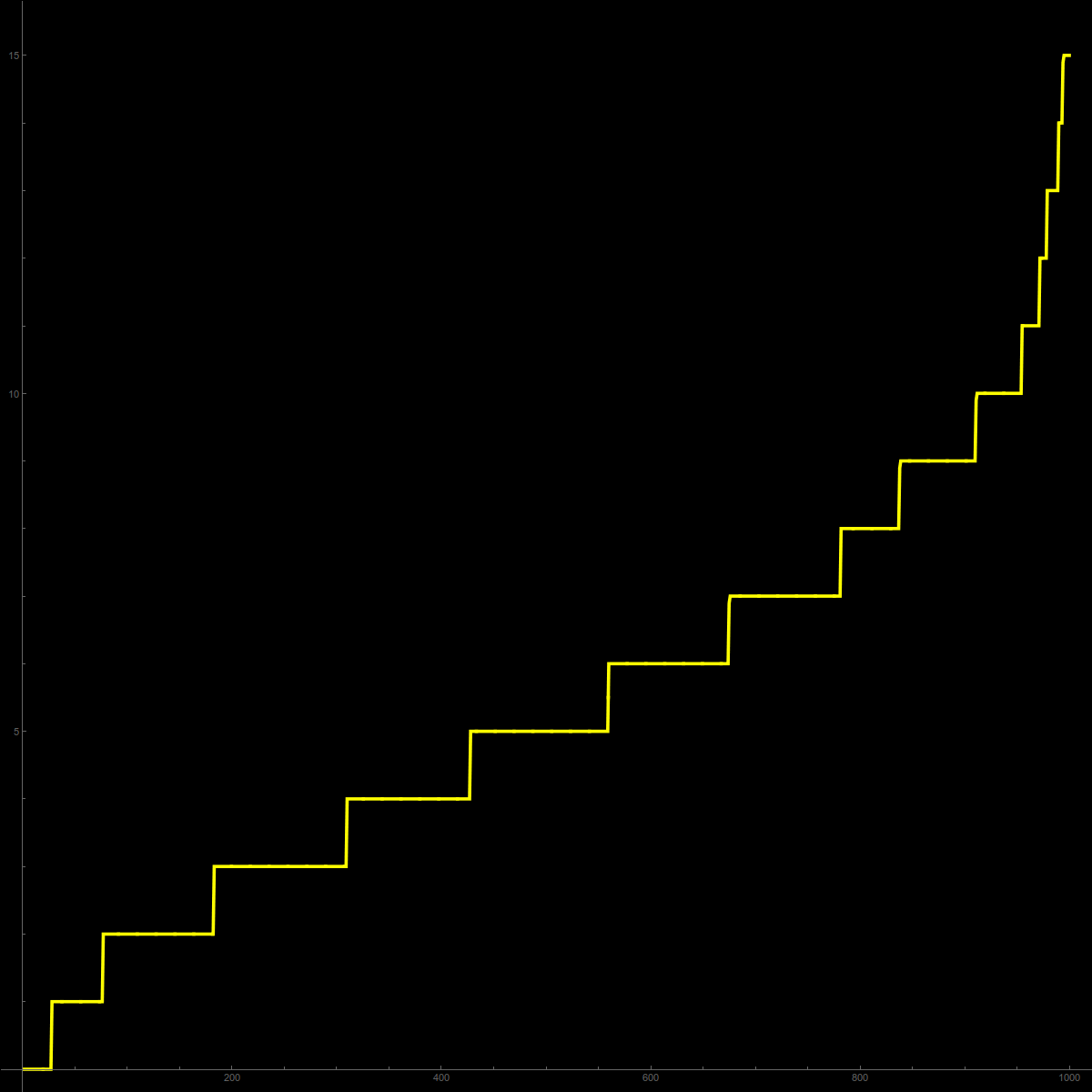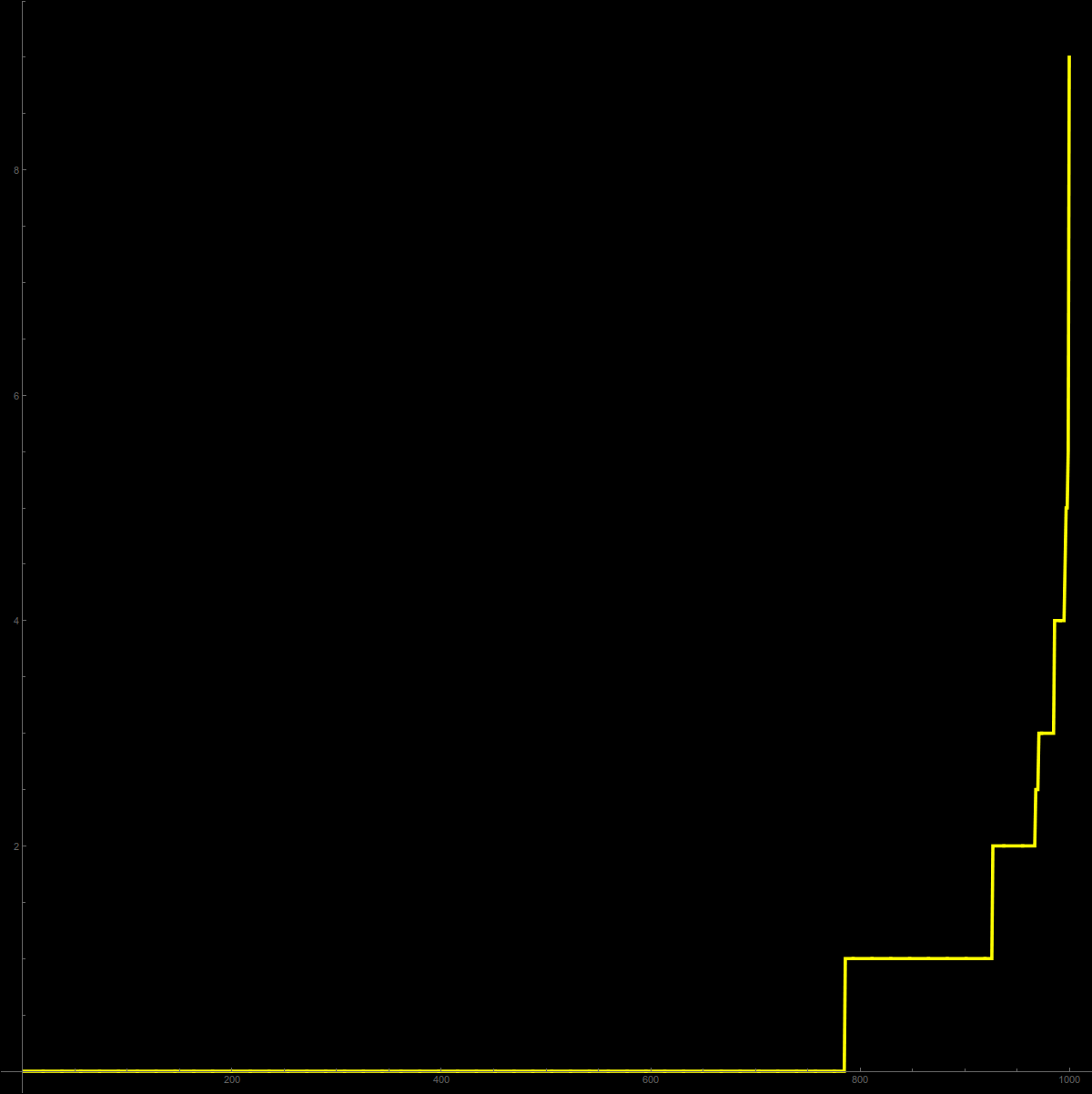Barycentric invariants 1-4 for 1000 Erdoes Renyi graphs E(12,0.5)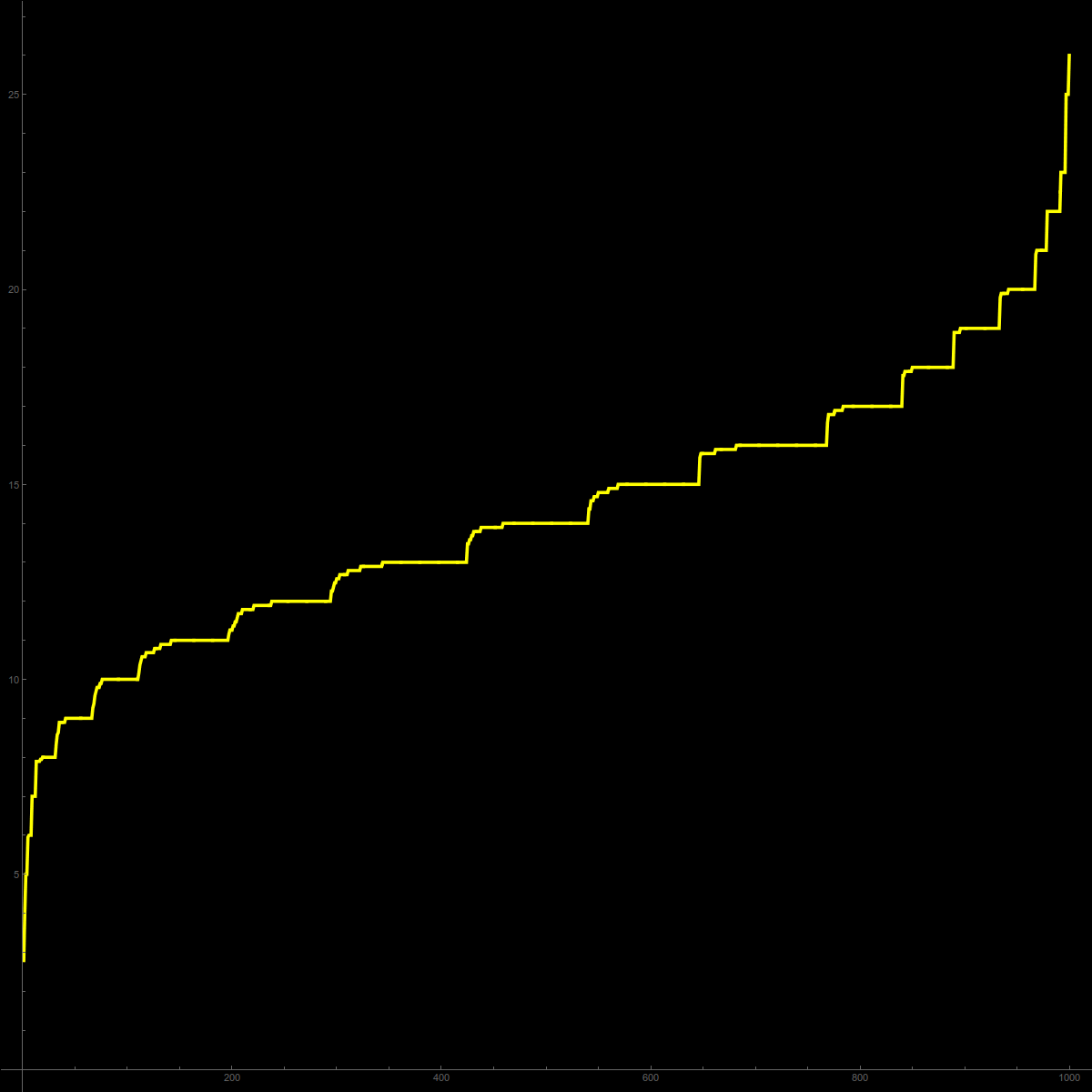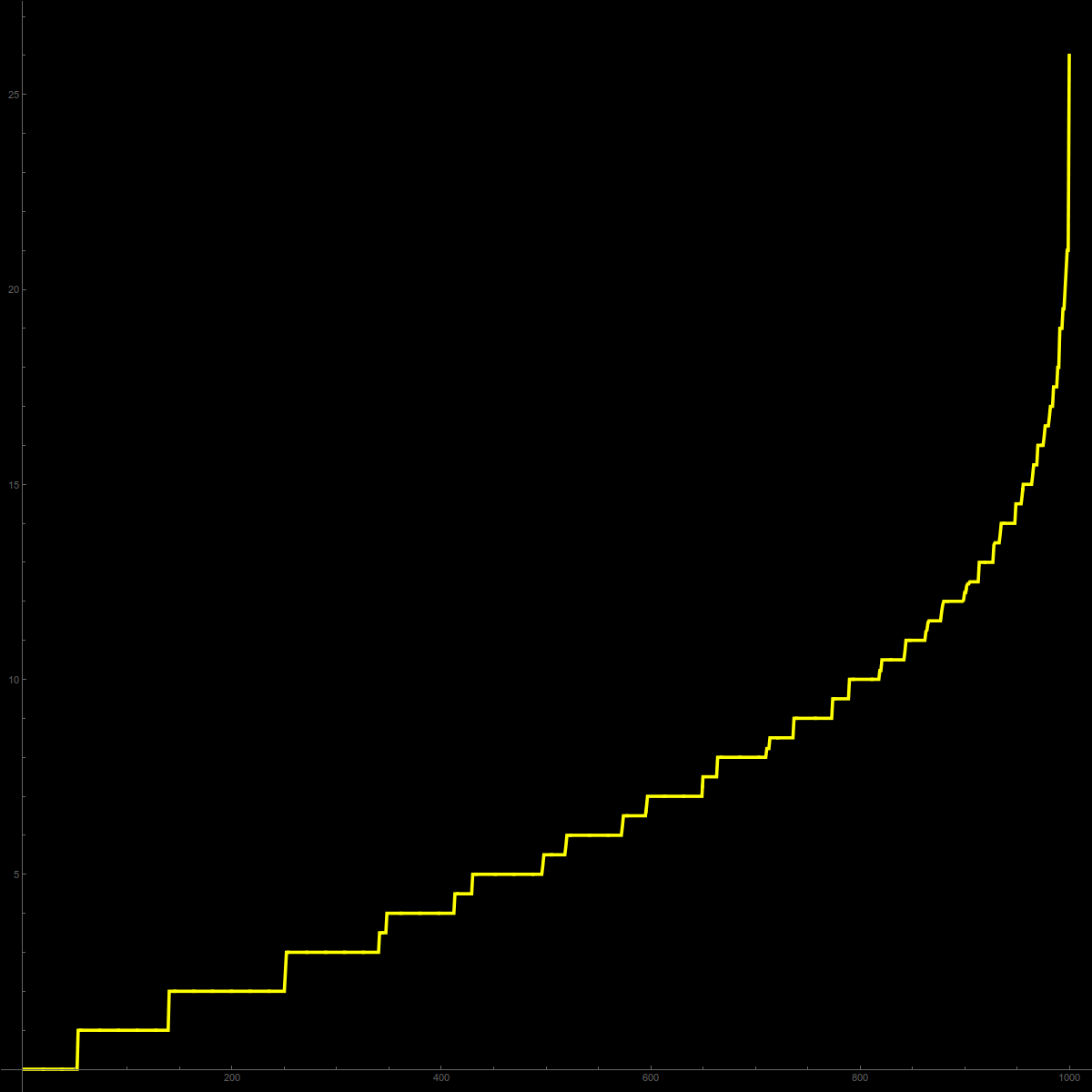• September 26, 2015: the picture is clear now: each Barycentric invariant Xk on a d-graph with k+d even is an average of Barycentric invariants of d-2 graphs, where we have a first average over the graph (Gauss Bonnet) and an average over functions (Poincare-Hopf averaged is Gauss Bonnet). Its the same (but generalized) story as for Euler characteristic for odd dimensional graphs (a special case), where curvature is zero proven here. Actually, what we did there already is establishing Dehn-Sommerville relations for spheres. Now, we have more general Klee-Dehn-Sommerville relations (with the same proof, much simplified due to Sard). So, here are the results: let G be an arbitrary finite graph: Parts B-D hold in general for any finite simple graph. Only part E needs the graph to be a discrete manifold:

 A) Klee-Dehn-Sommerville.: (will follow from B-E) Xk is zero if d+k is even. B) Gauss-Bonnet (done here in the Euler characteristic case k=1, also been found by Evako) There exists local curvature Kk(x) such that the vertex sum of K is Xk. D) Poincare Hopf (done here in the Euler characteristic case k=1. See also this exposition and a simpler proof) For locally injective function f, there exists an index if(x) such that the vertex sum of i is Xk D) Integral geometry ( here and here for colorings.) The average over all if(x) is K(x) when integrating over a reasonable probability space like the space of all colorings. E) Index relation (for k=1, done here and see also this discussion). If G is a d-graph, then if(x) can be written in terms of an invariant Xk applied to a (d-2)-graph.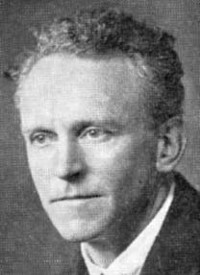Victor Klee Max Dehn (1878-1952) Duncan Sommerville (1879-1934)

The index formula parts generalize now nicely thanks to Sard. So, while I had been excited about discrete relations like tweeted here, it turns out that a whole theory exists which allows to recover it. So, while this result can be derived from known results in combinatorial topology, its proof outlined just above seems new. It actually appears also have more potential since one can also reach cases of discrete manifolds with boundary which appear quite algebraic and technical so far. The approach through Barycentric refinement also gives a direct path how one gets the Klee-Dehn-Sommerville invariants. They can appear otherwise to come out of the blue. Even if we would not know about Euler characteristic, we would be forced to consider it after seeing the Barycentric picture.
The story of invariants starts with Descartes and his discovery of the Euler characteristic. Euler gave the first proof shaped the invariant to become Euler's gem until geometers from Schläfli to Poincare pushed it to a topological invariant for manifolds. It was a painful story as the ``theorem" had to be adapted and extended as ``counter examples" emerged. Lakatos used it explain how mathematical theorems emerge in an evolutionary way. The Euler gem needed to be polished over some time. It is without doubt the most important invariant in topology. One of the problems was to define what a ``polyhedron" is. As Branko Bruenbaum once said: "Polyhedron" means different things to different people. Are there other invariants of this type using the clique numbers only? The Barycentric picture shows that the answer is "NO" if the invariant is linear in the f-vector unless the invariant is zero. The reason is that such an invariant has to be invariant under Barycentric subdivision and so an eigenvector of the Barycentric operator to the eigenvalue 1. But there are invariants which are zero. These are [(d+1)/2] quantities called Klee-Dehn-Sommerville relations. They span a linear space of such invariants. This linear space can be obtained directly from Barycentric subdivision too. Now, also this seems to be known already, but I definitely have a new proof for that which uses a notion of curvature. The path through Barycentric refinement shows that it is unavoidable. There is an other way to see that Euler characteristic is unique as Hadwiger has shown in 1955 that Euler characteristic on subsets of Euclidean space can be characterized by the fact that it is a valuation which is zero on the empty set and 1 on non-empty convex sets.
The Klee-Dehn-Sommerville relations were first discovered for for 4-polytopes and 5-polytopes by Max Dehn at the beginning of the 19th century. Duncan Sommerville generalized them in 1927 to convex d-polytopes. Victor Klee generalized it to combinatorial d-manifolds. The reason for the invariants is Poincare duality showing that half of the Betti numbers of such manifolds are redundant. Similarly, half of the clique data are redundant. The Klee-Dehn-Sommerville relations tell which. Now what happens is that the Barycentric refinement story gives an other approach, showing that the invariants are zero because their Gauss-Bonnet curvatures are zero. The approach even generalizes to combinatorial graphs with boundary, which seems to be already close to the state of the art, as Isabella Novik and Ed Swartz have shown a formula of this type in 2009.
One of the driving forces for the investigation in this area of combinatorial topology was the "upper bound conjecture" for simplicial d-spheres. The question was a very natural one means for graphs: What is the maximal volume of a d-sphere with n vertices? The question has first been formulated by Theodore Motzkin, proved in 1970 by Peter McMullen for polytopes and in 1975 by Richard Stanley for simplicial spheres who used new techniques like Cohen Macaulay graphs, graphs for which the face ring is a Cohen-Macauley ring.
• September 24, 2015: So, what could the Barycentric characteristics be used for?
1. One thing tried so far is to aim for inequalities for Betti numbers. For Euler characteristic, there is the elementary but beautiful Euler-Poincare formula which is just linear algebra (See lemma 4.4 in the fixed point paper). For general Barycentric invariants, the cancellations will not happen anymore, but one gets equations. Its possible that for suitable linear combinations of invariants, one gets enough cancellations to get nontrivial constraints for Betti numbers of large dimensional compact manifolds. In general however, it could be interesting to bound simplex numbers from the Betti numbers.
2. Since curvature adding up to Xk is either located in the interior or at the boundary of a d-graph with boundary, the invariants should be cobordism invariants. This is well known for Euler characteristic and trivial for volume as the boundaries have zero volume. It should hold in general. Now the question is of course, whether two manifolds are cobordant if all their Barycentric characteristics agree.
3. It is an old argument that a manifold M with odd Euler characteristic can not be the boundary of a larger dimensional manifold N. The reason is that 2 X(N)-X(M)=0 implies X(M) must be even. Now, the same can be done with the other invariants. Of course, one would need an example, where the Euler characteristic is even and an other Barycentric invariant can jump in.
• September 23, 2015: On the class of d-graphs, there are d+1 Barycentric characteristics Xk(G). Under Barycentric subdivision, the Barycentric characteristics Xkscales by the factor k! if a single Barycetric refinement is applied. The Barycentric characteristic X1 does not change under subdivision and is the familiar Euler characteristic. It always is a topological invariant. The k=(d+1)'th invariant is the volume. It is never an invariant: topological deformations of volume changes in general the volume. In this case k=d+1, the sum k+d is 2d+1 which is odd. An other trivial case is the d'th Barycentric invariant which is just a handshake result telling that twice the total number of maximal simplices is the sum of the maximal degrees summed over all vertices divided by d+1. As in the case k=1, where the Euler curvature integrates up to the Euler characteristic, there is a curvature Kk(x) on the vertices of the graph G such that its sum over all vertices is Xk. This is a generalized Gauss-Bonnet result:

 The sum over all Kk(x) over the vertex set is the Barycentric characteristic Xk(G) of the graph G.

This follows from the fundamental theorem of graph theory in the same way than Gauss-Bonnet. It can be explained with a few slides. As one can see, any linear functional on simplex data has an associated curvature localized at vertices such that the sum over all vertices is the total functional. Here is the new theorem about Barycentric characteristics:

 If G is a d-graph and if d+k is even, then Xk(G)=0.

[In the rewrite, the counting was from the other k -> d+2-k end so that X1 is volume. In that case, the invariants hold for all even k] This generalizes the fact that for odd d, the Euler characteristic X1(G) is zero (a fact which also more directly follows from Poincare-duality).
The simplest first not yet known case is the statement: A 4-graph containing v vertices, e edges, f triangles, g tetrahedra, p pentatopes always satisfies 22e+40g=33f+45p. The next example is A 5-graph containing v vertices, e edges, f triangles, g tetrahedra, p pentatopes and h hexatopes always satisfies 19f+55 p = 38 g + 70 h . Here are the numbers. The functional on graphs is always Xk(G)=vk.c(G), where c(G) are the clique data.
```
d=6  Barycentric characteristics
---------------------------------------------------------------------
k=7 v={0, 0, 0, 0, 0, 0, 1}         6-volume
k=6 v={0, 0, 0, 0, 0, -2, 7}        handshake
k=5 v={0, 0, 0, 0, 41, -123, 238}   not an invariant
k=4 v={0, 0, 0, -58, 145, -245, 350} gives zero invariant NEW
k=3 v={0, 0, 15941, -31882, 46145, -58730, 69881}  not an invariant
k=2 v={0, -7898, 11847, -14360, 16155, -17528, 18627} invariant NEW
k=1 v={1, -1, 1, -1, 1, -1, 1}      Euler characteristic
---------------------------------------------------------------------
d=5  Barycentric characteristics
---------------------------------------------------------------------
k=6 v=(0, 0, 0, 0, 0, 1)         5-volume
k=5 v=(0, 0, 0, 0, -1, 3)        handshake trivially zero invariant
k=4 v=(0, 0, 0, 58, -145, 245)   not zero in general
k=3 v=(0, 0, -19, 38, -55, 70)   gives a zero invariant      NEW
k=2 v=(0, 7898, -11847, 14360, -16155, 17528)   not zero in general
k=1 v=(-1, 1, -1, 1, -1, 1)      Euler characteristic is zero
---------------------------------------------------------------------
d=4   Barycentric characteristics
---------------------------------------------------------------------
k=5 v={0, 0, 0, 0, 1}            4-volume
k=4 v={0, 0, 0, -2, 5}           handshake
k=3 v={0, 0, 19, -38, 55}        not an invariant
k=4 v={0, -22, 33, -40, 45}      gives zero invariant        NEW
k=5 v={1, -1, 1, -1, 1}          Euler characteristic  Schlaefli
---------------------------------------------------------------------
d=3  Barycentric characteristics
---------------------------------------------------------------------
k=4  {0, 0, 0, 1}                3-volume
k=3  {0, 0, -1, 2}               handshake
k=2  {0, 22, -33, 40}            not an invariant
k=3  {-1, 1, -1, 1}              Euler characteristic is zero
---------------------------------------------------------------------
d=2  Barycentric characteristics
---------------------------------------------------------------------
k=3  {0,0,1}                     area
k=2  {0,-2,3}                    classical Euler handshake
k=1  {1,-1,1}                    v-e+f   Descartes
---------------------------------------------------------------------
d=1  Barycentric characteristic
---------------------------------------------------------------------
k=2  {0,1}                       length
k=1  {-1,1}                      1 minus genus = cyclomatic minus 1
is zero for geometric = circular G
---------------------------------------------------------------------
```
All these statements work for nice triangulations of smooth compact d-manifold, where "nice" means that the triangulation is a d-graph.

As there, the proof shows something stronger:

 The Barycentric curvatures Kk(x) are constant zero on G if d+k is even.

This was first discovered experimentally in the case k=1 and odd d in 2011 by computing many examples. In 2012, there was a proof using integral geometric technique by writing curvature as an average of Poincare-Hopf indices which themselves are written using the Euler characteristic of a (d-2)-dimensional graph defined at the vertex and the Euler characteristic of the unit sphere. Now, by induction, since the (d-2)-graph has zero characteristic and the unit sphere 2 (cancelling a term), the curvature is zero at the vertex.

The new result is proven in the same way. The induction assumption is that for odd d+k, the situation can be reduced to k=d+1, where we have volume, preventing the result. For d+k even, induction reduces the situation to k=d, where the handshake result holds.

How will induction work? For any locally injective function f (coloring) on the vertex set V, define a Poincare-Hopf index (divisor) as if,k(x) = X_k( { y | f(y) ≤ f(x) } ) - X_k( { y | f(y) < f(x) } ) Now almost by definition, the Poincare-Hopf theorem holds for these indices. They sum up to the Barycentric characteristic. Also, again as before, define the symmetric index jf = [if + i-f]/2. Now, again like before, the expectation of the index is the curvature. Now, since the symmetric index can be expressed as Barycentric invariants of smaller dimensional graphs, the result will follow by induction.
• September 20 evening, 2015} A complete rewrite of the Barycentric convergence story.

The proof of the statement that all even Barycentric invariants are zero appears indeed to be integral geometric: I needed a year to prove that the curvature K(x) is zero for odd dimensional graphs (a quantity which is not even defined in the continuum Gauss-Bonnet-Chern for odd dimensional manifolds as it involves a Pfaffian). The strategy (given here, see also here, and here) was to write curvature as expectation of Poincaré-Hopf indices, particularly important divisors introduced here to graph theory. [ This of course works also in the continuum, its just the other way round: take a probability measure on smooth Morse functions and define curvature as the expectation of the Poincare-Hopf indices. Then Gauss-Bonnet-Chern is trivial for that curvature as it is the expectation of Poincare-Hopf. As work of Banchoff has shown, one can get the usual curvature by taking Morse functions coming from linear functions in an ambient Euclidean space after Nash embedding the Riemannian manifold. We still don't have a natural intrinsic probability space on Morse functions (not using Nash embeddings) which produces curvature. A natural candidate are heat signature functions from the heat kernel in which the Riemannian manifold itself is the probability space. ] The recent Sard paper just substantially simplified the proof that curvature is constant zero for odd dimensional graphs as the symmetric indices [if(x) + i-f(x)]/2 can be written as the Euler characteristic of (d-2)-graphs. For odd dimensional graphs, one can prime the situation with d=-1, where we have only the empty graph which has Euler characteristic zero. It followed that curvature is constant zero for 1 dimensional 1-graphs (unions of circular graphs), and so Euler characteristic zero. For 3-graphs, the central manifolds Bfl(x) are one-dimensional with zero Euler characteristic so that the symmetric Poincare-Hopf index is constant zero. Hence the curvature, as an average of such indices, is constant zero for 3-graphs. Now we can proceed to 5-graphs. Again the curvature is the expectation of Euler characteristic of 3-graphs which therefore must be zero. Etc. Generalizing this to Barycentric invariants is only natural. We aim is now to prove that in any dimensions d (my original restriction to even dimension is not necessary)and every even eigenvector fk of AT, the corresponding invariant is zero for all d-graphs. In the odd-dimensional case, since then the Euler characteristic is an even eigenvector, this includes the already known fact that the Euler characteristic is zero for odd-dimensional graphs (which as in the continuum also follows from the Poincare duality). So, it is clear what to do: introduce for every Barycentric functional Xk a curvature Kk(x) on the vertex set and write the Barycentric invariant as an expectation of generalized Poincare-Hopf indices i(f,k)(x) in the same way in the case k=d+1, where we deal with the usual curvature for Gauss-Bonnet-Chern for graphs. Now show that the symmetric Barycentric Poincare-Hopf indices j(f,k)(x) defined as before are Barycentric invariants in dimension d-2 for an eigenvector labeled k-2. The primer is the handshaking invariant k=2 which is a zero invariant for all d-graphs. Now, this still has to be written down. But the basic ingredients are the same like that like Euler characteristic, any of the Barycentric invariants have the property X(A v B) = X(A) + X(B) - X( A ^ B) known for valuations. In the discrete, this is of course trivial, since each of the clique data vk has this counting property. The story does not end. Once we know that what the indices are, we are eager to look what these divisors mean in the continuum limit. As Poincare-Hopf divisors, they should be defined for any Morse function f.
• September 20, 2015: Here are the Barycentric invariants for 8-graphs
```k=1 (0,0,0,0,0,0,0, 0,1)                  volume
k=2 (0,0,0,0,0,0,0,-2,9)                  handshake
k=4 (0,0,0,0,0,-22,77,-168,294)           B2
k=6 (0,0,0,-97382,243455,-411355,587650,-765492,941325)   B4
k=8 (0,-159215782, 238823673,-289483240,325668645,-353346952,375501693,-393818080,409332165)
k=9 (1,-1,1,-1, 1, -1, 1, -1, 1)          Euler characteristic
```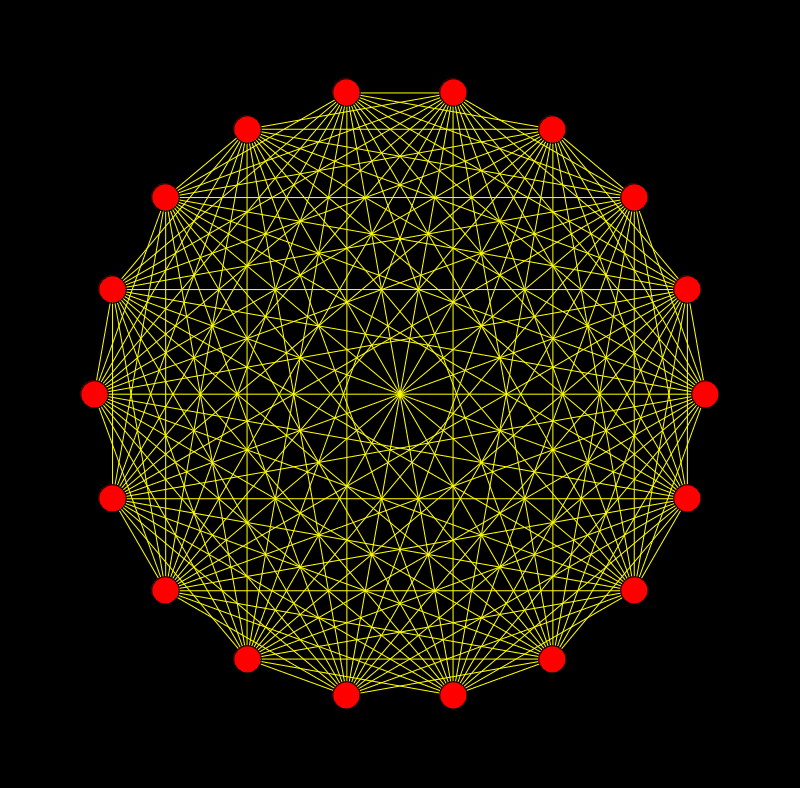Lets look at at an 8 dimensional sphere G (the 8-dimensional octahedron). The graph is shown in the picture. You see why graphs are nice. Unlike simplicial complexes which need a considerable amount of math to even be defined, any kid can draw an 8 sphere: draw a circle, place 18 points and connect any two dots, except dots which are opposite to each other. This is an 8 dimensional Evako sphere: each unit sphere is a 7 sphere. This 8-sphere has the clique data
```v(G) = (18, 144, 672, 2016, 4032, 5376, 4608, 2304, 512)
```
and of course Euler characteristic 2 like any even dimensional sphere. It is perpendicular to the eigenvectors vectors B2,B4,B6. Again, under edge refinement operations, this remains true. One can show this algebraically but here is an example, where one random refinement, we end up with a graph having clique data v(G) = {19, 159, 770, 2380, 4872, 6608, 5728, 2880, 640}. The edge refinement has changed the clique data by adding {1, 15, 98, 364, 840, 1232, 1120, 576, 128} which (when ignoring the first entry) are the clique data of the 7-sphere S(x), where x was the added vertex splitting the edge.

I believe that given any two d-spheres G,H in the Evako sense (defined in detail in the Jordan paper), there exists a finite set of edge or Barycentric refinements or inverse operations so that the two end graphs are isomorphic. Sounds a bit like the Hauptvermutung but it must be much easier as we are in a completely finite combinatorial setting and have a class of triangulations which are completely controlled. Triangulations in topology are difficult and messy (there are even many different definitions), but if we restrict to triangulations which are d-graphs, this is easier as we have control about the unit spheres and any finite intersection of unit spheres, as they are all smaller dimensional spheres. Having this recursive "niceness" makes proofs easier as we can proceed by induction in dimension. That became evident already when looking at Jordan-Brower.

More generally, for any two graphs G,H which are homeomorphic in the sense defined here one should be able to deform them into each other using edge refinements and Barycentric refinements. If this is true, the invariant needs only to be verified for one element in its topological class.
• September 19, 2015: Why are Barycentric invariants interesting? Its because they vanish for discretizations of differentiable manifolds. They do not disappear for graphs with uniform dimension 2d, graphs for which the unit spheres are (2d-1) dimensional graphs which are not necessarily spheres. One could imagine for example that some unit spheres are discrete projective spaces or tori. As I had speculated for example here there is a chance that some exotic 2d-graphs exist, graphs which are homeomorphic to a 2d-graph but which are not actual 2d-graphs. For such a graph, the Barycentric invariant is not zero (there is no reason for it to be). The situation is more subtle than in the case of discrete algebraic sets where already the handshaking invariant allows to figure out that a graph is not a discrete manifold. Assume we had such an exotic graph G, homeomorphic to a 2d-graph H, then also the Barycentric refinements are homeomorphic. It follows that also the limiting manifolds are homeomorphic. Now comes the punch line: the limiting manifolds can not be diffeomorphic because for diffeomorphic manifolds, integer valued integral geometric invariants must agree. The linear space of integral geometric invariants in the continuum and discrete are of the same nature, have the same dimension and can with a generalized setup be treated in a uniform way. So here is what likely going to happen: Let f0,....,f2d be a Hadwiger basis for d manifolds in the continuum. For every of the Barycentric graph invariants which is zero for 2d-graphs, there exist constants a0, ....ad such that the sumk ak fk is an integer, which is zero for all differentiable d-manifolds. For Barycentric refinement of exotic graphs for which the invariant is not zero, also the scaled limiting invariant is not zero. The graphs are homeomorphic, but not diffeomorphic and the integral geometric invariant should see that. P.S. Just to be clear about the tweet:
```A triangulation of a compact 4-manifold
containing v vertices, e edges, f triangles, g tetrahedra,
h pentatopes satisfies  22e+40g=33f+45h
```
There is no proof yet why this is true in general for any smooth 4-manifold. If there should be 4 manifolds M, for which X(M)=22e-33f+40g-45h is not zero, then this would of course be even more exciting. As just pointed out, X(M) is likely not zero for exotic manifolds like E8 which are equipped with unusual differentiability structures. [Added September 20: At the moment, it looks as if X(M) vanishes for smooth or PL manifolds (but this is only intuition from integral geometry). A first step will be to prove a completely finite combinatorial result that X vanishes for all 4-graphs.]
• September 18, 2015: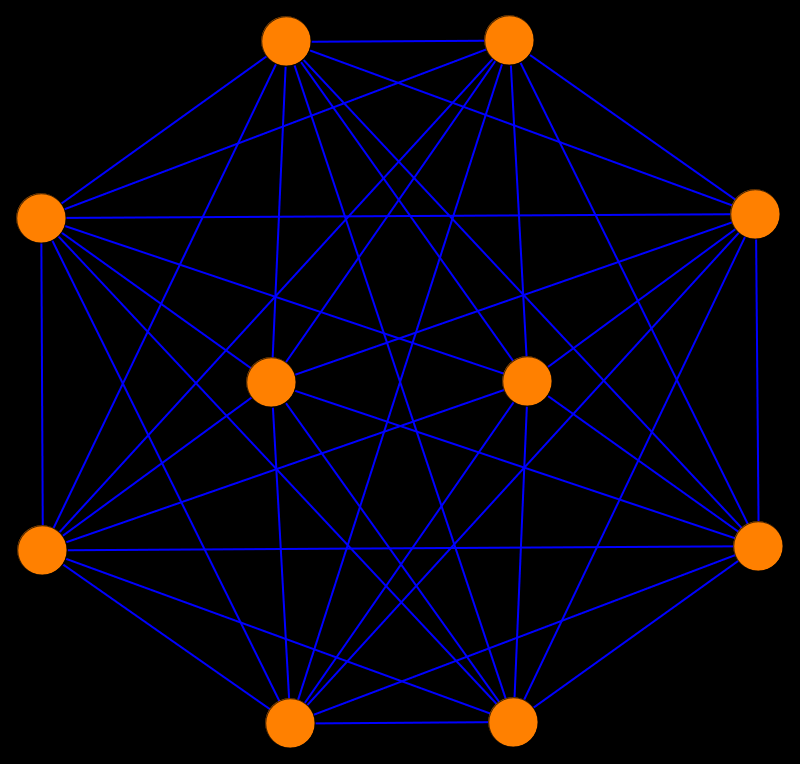Very exciting: for even dimensional 2d-graphs (graphs for which the unit spheres are (2d-1)-spheres), the eigenfunctions f2k of the Barycentric operator AT lead to new invariants. The first new case is for 4-graphs = discrete 4 manifolds which appear for example for nice triangulations of real 4 manifolds. Lets take a 4-sphere: with clique vector v=(10,40,80,80,32). The eigenvectors of AT restricted to 4-graphs are (0, 0, 0, 0, 1),(0, 0, 0, -2, 5),(0, 0, 19, -38, 55), (0, -22, 33, -40, 45),(1, -1, 1, -1, 1), where the first is volume and the last is the well known Euler characteristic. The second and fourth vectors are perpendicular to the clique vector.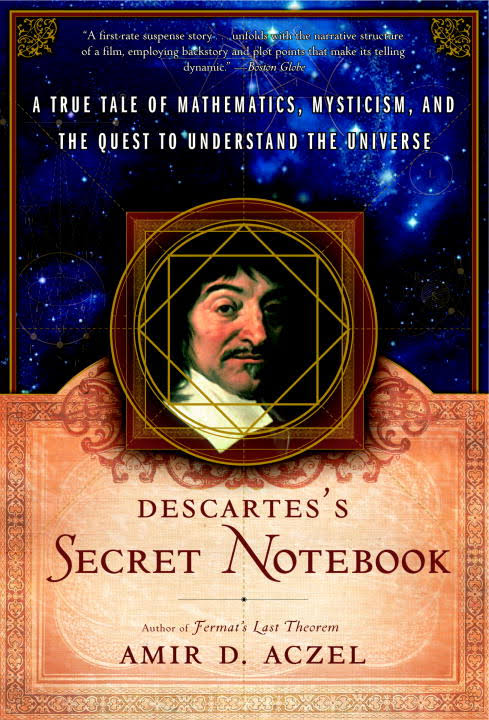The second is the trivial handshake invariant. The fourth leads to a new Barycentric invariant which I have never seen:
 X(G) = -22 v1 + 33 v2 - 40 v3 + 45 v4

I call it Barycentric invariant (the Euler characteristic is one too). By the way we are here in the stage of Descartes discovering the invariant v-e+f, which Euler first showed to be real but was only really understood with maybe Schlaefli or Coxeter, after many wrong turns and refutations. See the Lacatos book. There is of course a big difference between the time of Descartes and today. Descartes had no computers and had to fear plagiarism so that he wrote his findings in an encrypted way. Today we have powerful computer algebra systems for experiments, we blog and tweet everything (even premature results) right away.This functional on 4-graphs remains zero also after edge subdivisions (which I used for graph coloring embeddings). For example, after 10 random edge refinements, we get a deformed 4-sphere with data (20, 115, 280, 305, 122). It is perpendicular to (0, -22, 33, -40, 45) leading to X(G)=0. Also the clique data of the Barycentric refinement G1 which are (842, 9840, 30960, 36600, 14640) satisfies this by definition. The invariant appears to be zero for all 4-graphs independent of its topology. Here is an other example graph G=S2 x S2 with Clique data of G are (196, 2304, 7296, 8640, 3456) and Euler characteristic chi(G) = 196 - 2304 + 7296 - 8640 + 3456=4. We see that the graph product is handy for constructing examples. Before I had the graph product, I had to construct such examples by hand, now its for free. Also here, the mystery invariant is zero: X(G)=0. More examples:
• a discrete S2 x T2 with clique data v(G) = (1664, 23424, 77056, 92160, 36864) and after 25 edge refinements v(G) = (1689, 23689, 77866, 93110, 37244).
• a non-orientable 4-graph G=P2 x S2 with clique data v(G) = (1908, 26520, 87020, 104010, 41604) (and Euler characteristic 2, as it has to be as the projective plane has Euler characteristic 1 and the sphere Euler characteristic 2). An example after refinements (1923, 26703, 87602, 104700, 41880).
• A 4-torus with clique data v(G) = (1306, 19562, 65188, 78220, 31288) or after some refinements v(G) = (1321, 19709, 65626, 78730, 31492)
Once it is zero, it remains zero for all edge or Barycentric refinements or inverse operations. We suspect that one can get this every graph homeomorphic and so prove that X(G)=0 for all 4-graphs. For 6-graphs, discrete 6-manifolds, there are two new invariants because the eigenvectors of the Barycentric operator are
```     (0, 0, 0, 0, 0, 0, 1)              volume
(0, 0, 0, 0, 0, -2, 7)             trivial boundary part
(0, 0, 0, 0, 41, -123, 238)
(0, 0, 0, -58, 145, -245, 350)              NEW: on 4-graphs
(0, 0, 15941, -31882, 46145, -58730, 69881)
(0, -7898, 11847, -14360, 16155, -17528, 18627)   NEW: zero on 4-graphs
(1, -1, 1, -1, 1, -1, 1)           Euler characteristic
```

 X(G) = -7898 v1 + 11847 v2 - 14360 v3 + 16155 v4 -17528 v5 + 18627 v6 Y(G) = -58 v3 + 145 v4 -245 v5 + 350 v6

For a discrete 6-sphere with clique data (14, 84, 280, 560, 672, 448, 128), and Euler characteristic chi(G) = 2, we have X(G) = 0 and Y(G) = 0. Again, these new functionals on 6-graphs lead to invariants.
• September 18. More Chladni pictures: cylinder and a larger square.
• September 15, 2015: Why is the Euler characteristic given by the alternating sum? We have now a natural answer:
 It is an eigenvector to the eigenvalue 1 of the Barycentric operator AT!

Since it is an eigenvector to the eigenvalue 1, it has to be an invariant also in the continuum limit. What about the other eigenvalues. This is extremely interesting. There is of course the eigenvector [0,0,0..,1]T on d-dimensional graphs which is the eigenvector to the eigenvalue (d+1)! It is the Volume. It is not an invariant but it scales under Barycentric refinement. But we can hope for more invariants. The eigenvector [0,0,....,0,-2,d+1] to the eigenvalue d! is a boundary volume term growing like d! but for d-graphs, graphs for which the unit spheres are d-spheres it become sn invariant because it is zero. This is a handshaking argument: three times the number of triangles in the d=2 case for example is 2 times the number of edges. Or in the d=3 case, 4 times the number of tetrahedra is 2 times the number of triangles. While Euler characteristic and this handshake invariant were well known, we have now a way to find them (if they were not yet known). But they have to be restricted to certain classes of graphs.
• September 14, 2015: finally got the code together to compute the matrix A, such that if v is the clique vector of a graph G, then A v is the clique vector of the graph G', the Barycentric refinement of G. Here is the Barycentric matrix A:
 1 1 1 1 1 1 1 1 1 1 1 ... 0 2 6 14 30 62 126 254 510 1022 2046 ... 0 0 6 36 150 540 1806 5796 18150 55980 171006 ... 0 0 0 24 240 1560 8400 40824 186480 818520 3498000 ... 0 0 0 0 120 1800 16800 126000 834120 5103000 29607600 ... 0 0 0 0 0 720 15120 191520 1905120 16435440 129230640 ... 0 0 0 0 0 0 5040 141120 2328480 29635200 322494480 ... 0 0 0 0 0 0 0 40320 1451520 30240000 479001600 ... 0 0 0 0 0 0 0 0 362880 16329600 419126400 ... 0 0 0 0 0 0 0 0 0 3628800 199584000 ... 0 0 0 0 0 0 0 0 0 0 39916800 ... . . . . . . . . . . . ...
• September 12, 2015: the operator AH belonging to the transformation mapping a graph G to G x H is an unbounded operator on the Hilbert space l2. When restricted to graphs of dimension d, it is a finite matrix. It maps the simplex data (v0,v1,...) counting the number of vertices, edges triangles, tetrahedra etc in G to the simplex data of the graph product G x H. What is the analogue of A for compact Riemannian manifold H? We could look at the valuations of M and map it to the valuations of M x H. As for graphs, we just can count to find the matrix, in the continuum, we would need to do some integral geometric computations and hope that A is an integer matrix if the length scale on M is chosen properly. While working with Barry Tng [Barry's thesis[PDF] on integral geometry, we have explored also the possibility to prove Hadwigers theorem by discrete approximations but there was no time to push it through. Anyway, the question to identify the linear map from valuations of M to valuations of M x H seems never been asked. As in the graph case, we can probe the map by doing examples. For example, if H is the circle, now compute the valuations M x H for M=two sphere, M=torus, examples of genus g surfaces. The map is linear because blowing up M by a linear factor obviously blows up M x H in the same way. As in the graph case, the top valuation (volume) is easy.
• September 12, 2015: As visible in the first draft, there was a struggle to find a formula for the number of Kk graphs in the Barycentric refinements. In order to tackle the d=3 dimensional case, we know that the number of tetrahedra grows like 24m. To count the number of triangles in the next step, we can multiply the old number by 24 and then compensate the double count at the boundaries. Similarly as for the recursion for the triangle
```u[m_]:={Total[u[m-1]],Total[u[m-1]]-1+6 u[m-1][],6*u[m-1][]};
u[0_]:={3,3,1}; u
```
there is first a recursion for the tetra boundary refinements
```v[m_]:={Total[v[m-1]],Total[v[m-1]]-2+6 v[m-1][],6*v[m-1][]};
v[0_]:={4,6,4}; v
```
This tells for example that for G=K4 the boundary of the ball G6 obtained by doing 6 times a Barycentric refinement of G is a graph with 93314 vertices, 279936 edges and 186624 triangles. This can now be used to crack the task of finding the clique data of the refinements of the graph K4. The recursion uses the function v defined recursively above:
```w[0_]:={4,6,4,1};
w[m_]:={Total[w[m-1]],
Total[w[m-1]]-1+24*w[m-1][]-12*v[m-1][]+v[m][]/2-24*w[m-1][],
24*w[m-1][]-12*v[m-1][]+v[m][]/2,
24*w[m-1][]}; Table[w[k],{k,0,5}] //MatrixForm
```
giving
```     4           6          4          1
15          50         60         24
149         796        1224       576
2745       17000       28080     13824
61649      396016      666144    331776
1455585    9433760    15940800   7962624
```
When done in this way, the case d=4 looks more complicated as we first to tackle the problem of finding a recursion for the boundary 3-spheres of the four dimensional balls Gm with G=K5, then use this to get the number of tetrahedra. To get the number of triangles, we have however to take into account how many hypertetrahedra intersect in such a triangle to compensate the over counting. This looks too complicated. Even if we succeed in the case d=4, we still don't see a recursion in general. Lets start all over: since the recursion map mapping the simplex data v = (v0,v1,...) of G to the simplex data of the refinement is linear, why not just try to find that linear map? Here is a bolder question: wouldn't it be nice, if in general, for any graph G with simplex data v, the Barycentric refinement G1 had simplex data w=Av which depend in a linear way on v and that A is independent of the graph G? It came to me as a surprise that this is actually true and the matrix A can be found:
```   1  1  1  1   1 ...
0  2  6 14  30 ...
0  0  6 36 150 ...
0  0  0 24 240 ...
0  0  0  0 120 ...
.. .. .. .. ..  ...
```
Since it applies for all graphs, it can be constructed by bootstapping up. NO need to distinguish between boundary and interior. So, to get the next (n+1)th colum, just apply the nxn submatrix to the vector (B(n+1,2),B(n+1,3)....,B(n+1,n)). Cool! For example, in order to find the new number of triangles in the barycentric refinement, we know that each of the old triangle spans 6 triangles. But additionally, each tetrahedron will produce 36 new interior triangles etc. Since Barycentric refinement is just a special case of an operation G -> G x H on graphs, where H=K1 and x is the graph product, we can generalize: there exists for every finite simple graph H a matrix AH such that the simplex data w of T(G) are w=AHv. In the case K2 already, the matrix is no more upper triangular:
```     3    3    3 ...
2   12   32 ...
0    8   66 ...
0    0   36 ...
--  --   --
```
As pointed out in here, the product T(G) is only associative if we do the multiplication on the algebraic level in which case K1 is the zero element, but when returning to the geometry, G x K1 becomes the Barycentric refinement of G.
• September 7, 2015: it is reasonable to believe that the spectral gaps are are related to severe topological changes of the Chladni figures, the level surfaces of the eigenfunctions defined in the Sard paper. The Chladni figures or nodal surfaces separate the nodal regions of the graph. Here are pictures of a triangle refinement.
• September 7, 2015: An other observation: the functions F(Gm) seem have the property F(Gm) ≤ F(Gm+1) in the case G=K3. Something we also can not prove yet. But it might also be subtle as in the case d=3, with G=K4, the monotonicity fails. Here are pictures showing some of the graphs of F(Gm) simultaneously: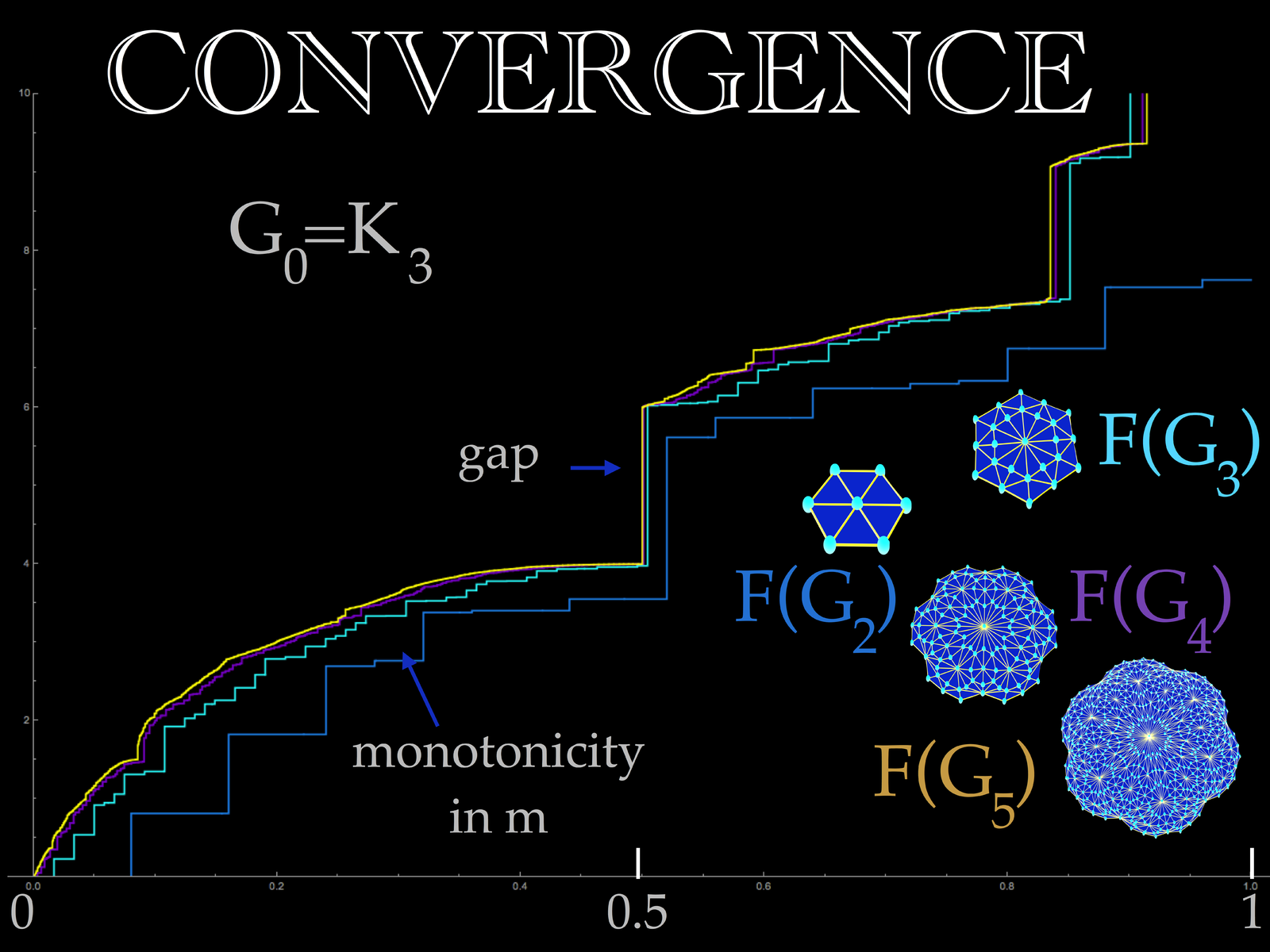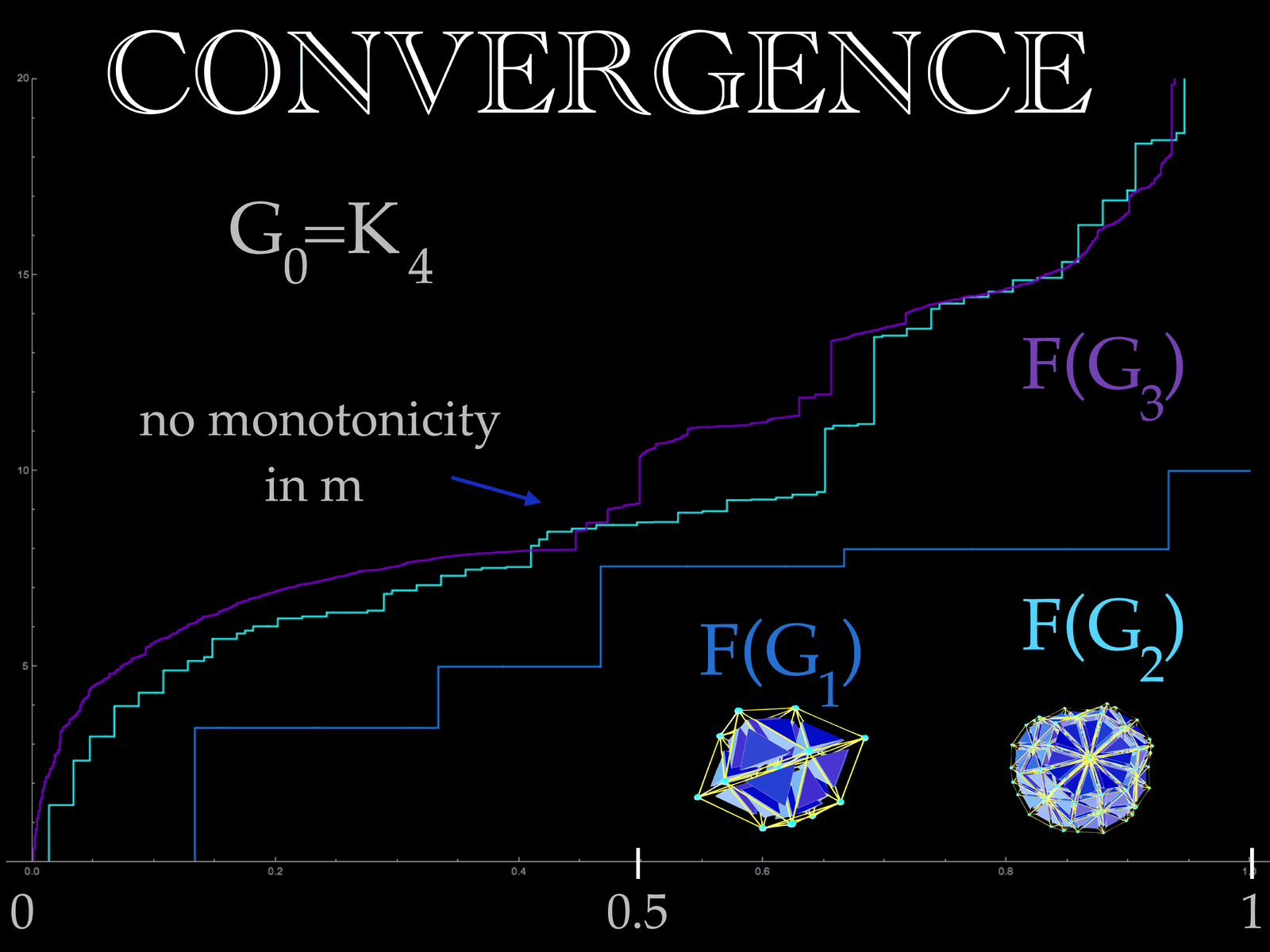• September 5, 2015: where are the jumps in the distribution in the case d=2, where Barycentric refinements are done on a triangle G? The graph G5 already has 3936 vertices. Here are the largest eigenvalue differences and candidates for gaps in the limit:G4 for the triangle G=K3. ```x F(x+epsilon)-F(x) ---------------------------- 0.5 2.002 0.835366 1.67669 0.917683 2.86249 0.972561 3.89394 0.98628 6.93379 0.995427 7.96794 0.998476 14.9767 ```
The spectral gaps seem to happen at places close to 1-2(-x) and the jump values appear to grow exponentially. A first challenge is just to estimate the limiting jump at x=0.5 in the limit. [September 6: starting with the triangle the L1 error is sum_k=3^infty 8/(d+1)k=4/9. There is a spectral gap for G2 which we can rigorously show to be larger than 2 (as the eigenvalues can be given as roots of polynomials of degree 4 in that case). It is unlikely that with an area change of less than 0.5 it will be possible to bridge the gap of 2. In order to verify the existence of the gap, we would need to be able to estimate the convergence on subintervals which is not unreasonable. There is hope that one can rigorously establish a spectral gap if we could have a Lidski type estimate for sub ranges of the spectrum.] But what is the reason for the spectral gaps? An intuitive explanation would be nice. It certainly should be dynamical using heat or wave dynamics. Or then with the Courant nodal theorem which gives a reason at which energies it will be possible to have nodal curves with small diameter. Lets look at the distribution of the difference F'(x), the differences of the eigenvalues. If you want to have a look, the file u5.txt (contains the list of eigenvalue differences) in the case of the triangle refinement. Below is a logarithmic plot obtained with ListPlot[Log[u],PlotRange->All]. We see that there are two regimes. In one, the differences are of rounding error size indicating repeated eigenvalues leading to "plateaus", then there is a range, where the difference is of the order expected for a continuous function. And then there are jumps. The above list shows the values with eigenvalue jumps bigger than 1. Experimental results indicate a devil stair case type function in the limit indicating point spectrum.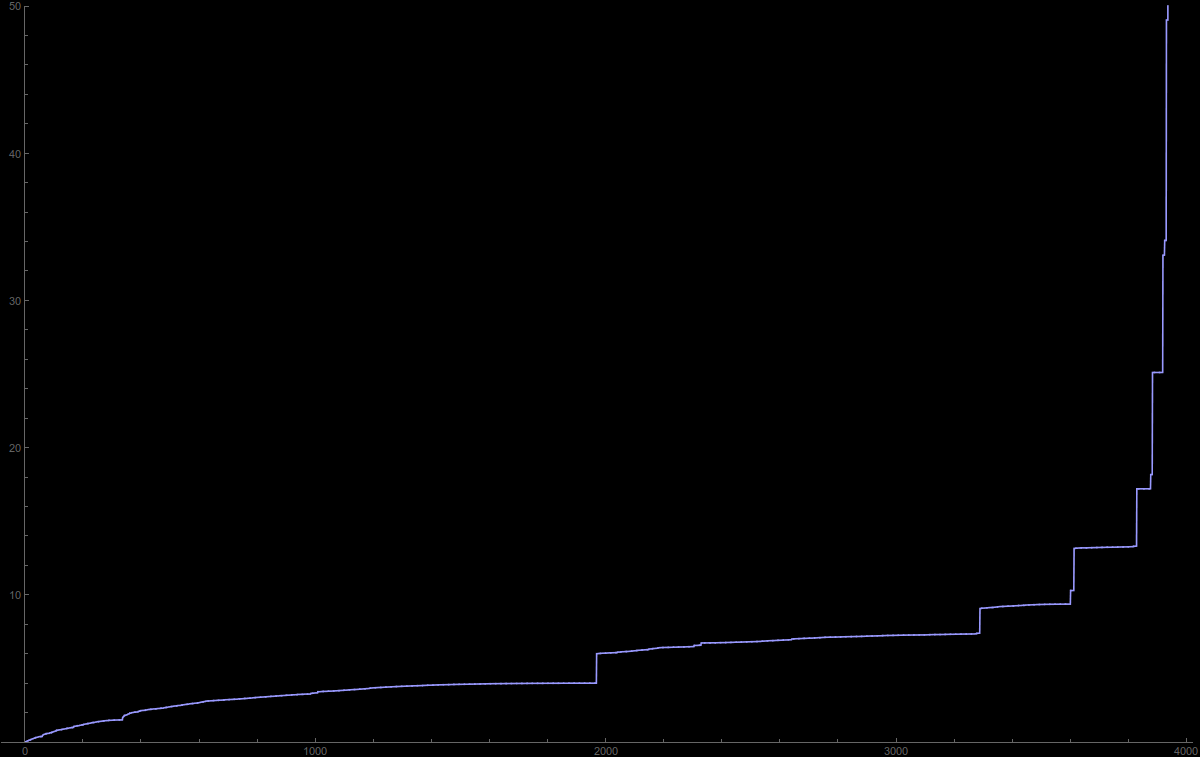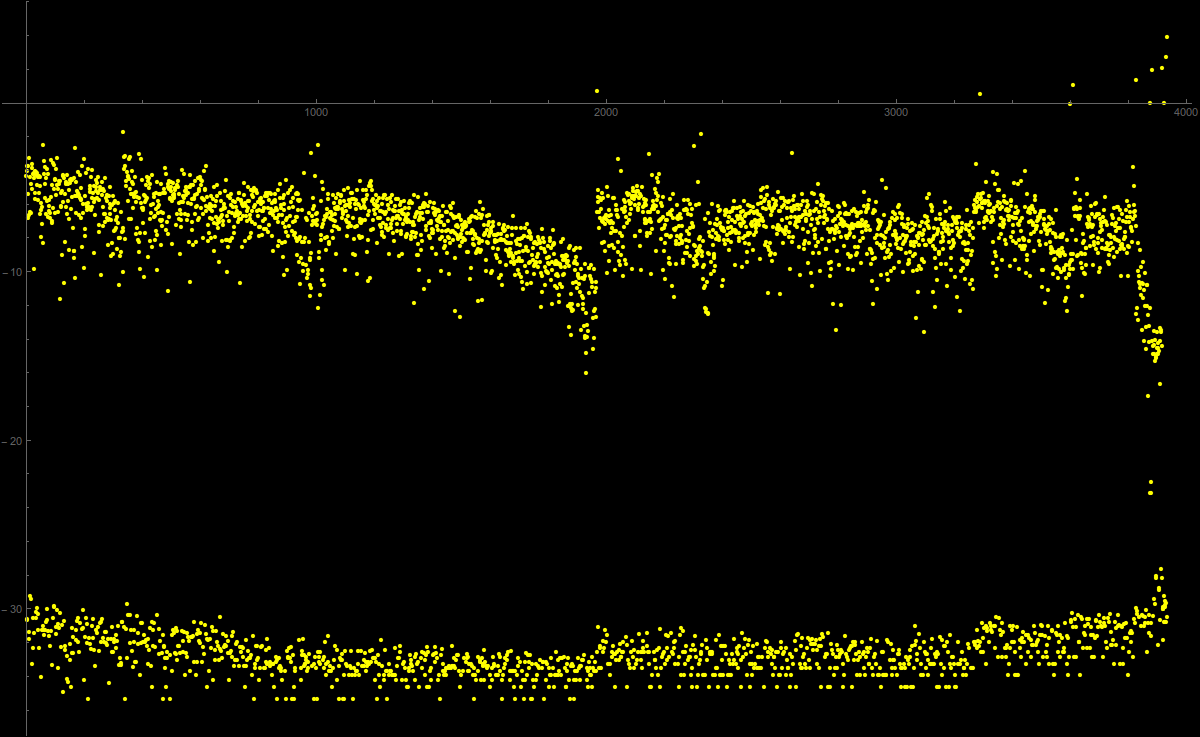Eigenvalues of G5 for G=K3 The logarithm of eigenvalue differences of G5 for G=K3.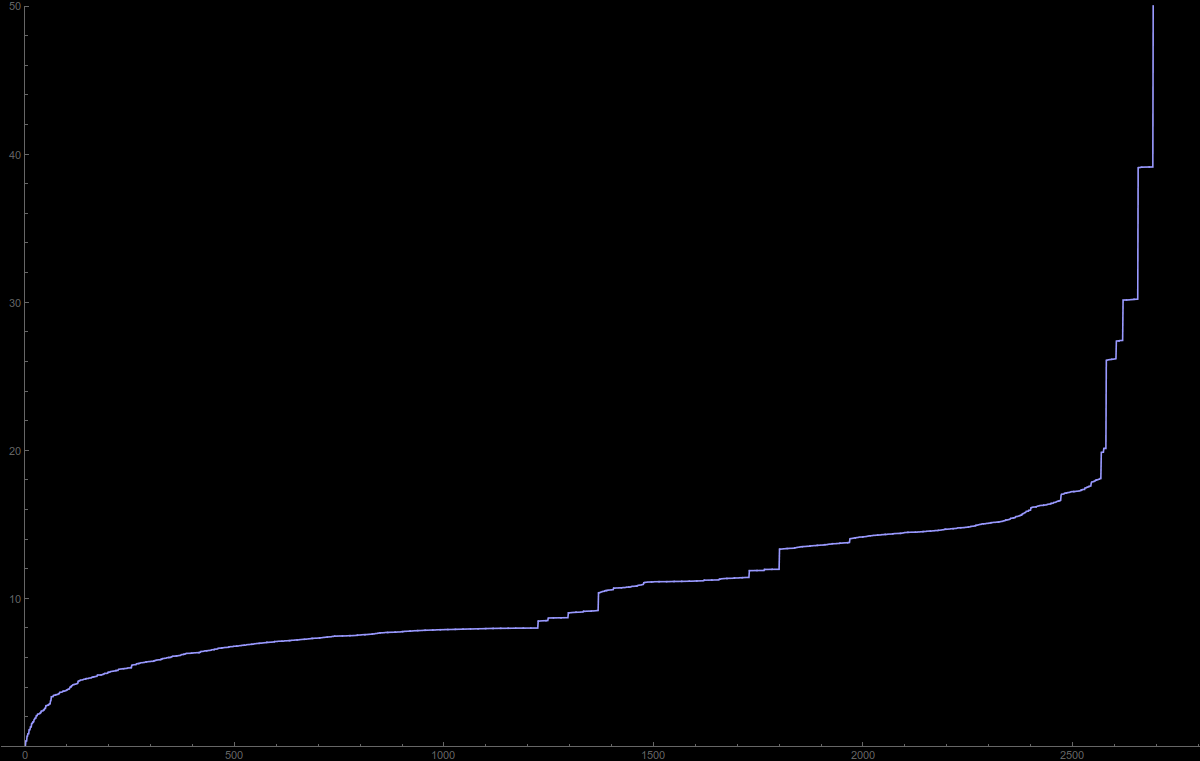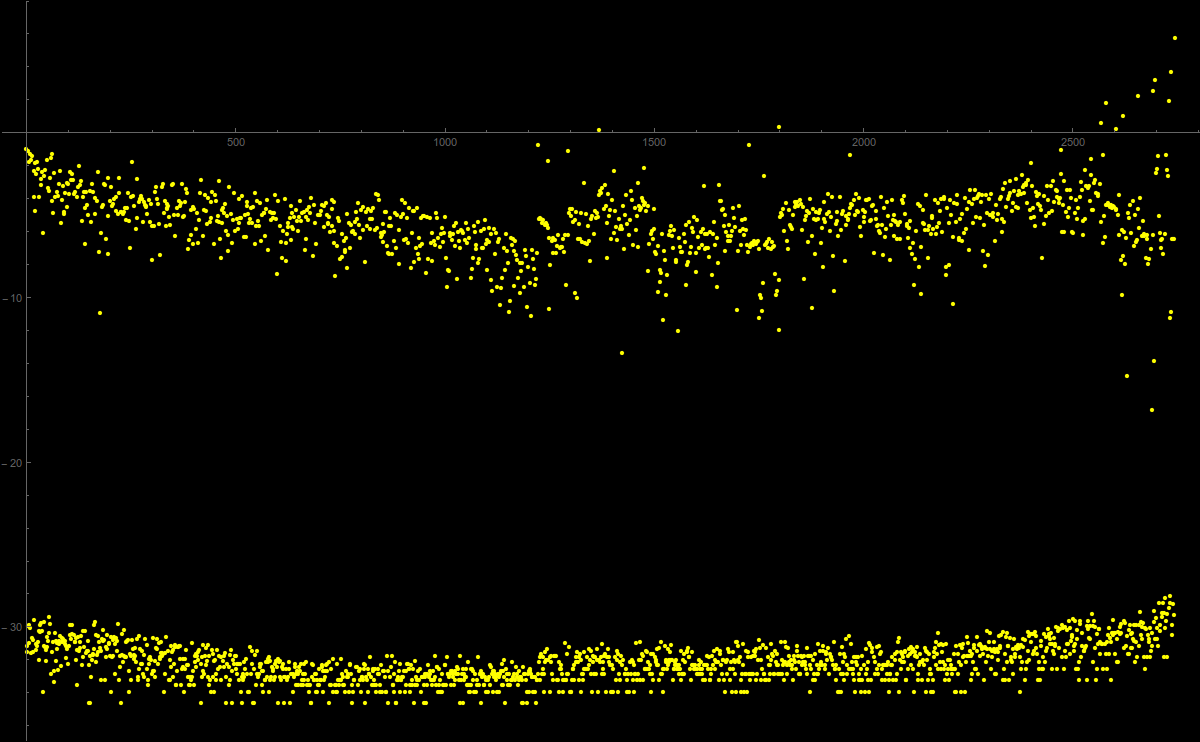Eigenvalues of G3 for G=K4 The logarithm of eigenvalue differences of G3 for G=K4.
• August 26, 2015: the graph software GraphTea has implemented a Barycentric refinement, but in the usual way graph theorists look at it, by just subdividing the edges. This produces homeomorphic graphs in the classical sense. If done like that, then after one subdivision, there are no triangles any more and the limiting spectral density is the one for d=1. It is usual in graph theory that only zero and one dimensional simplices are counted and not the entire Whitney complex.
• August 25, 2015: also in higher dimensions, the limit should be given by a "random operator" which is realized on a compact topological group. The terminology "random operator" is the same than for a "random variable": it is a matrix valued function on a probability space. Random operators over some dynamical system are elements in a nonabelian cross product C* algebra. There does not need to be any independence of the matrix entries. Actually the matrix entries of the limiting operator are probably almost periodic in the same way as in one dimension. Currently, I expect that there is in any dimension a compact topological group G and an action of a nonabelian (hopefully finitely presented) group A of translations on G such that the limiting operator L is of the form sumi Ui + i Ui*, where Ui are unitary generators. If the picture in one dimensions is an indication and if we see the Ui as translations on the group, then there is an other operation T, the chaotic renormalization map which rescales G and which is not invertible. This operation T plays the role of the shift in the one dimensional case where G is the compact topological group of dyadic integers. In one dimensions, the space translation is almost periodic while the rescaling map is Bernoulli. The prototype of such a situation but unrelated is G=T, the circle, where T is the map T(x)=2x and translation is a rotation U(x)=x+a mod (1). What is the group G? If the picture in one dimension is a good indicator, it is the product of automorphism groups of refined spheres. Each of these factors is a discrete subgroup of SO(d) and thats the reason why the group G is expected to be non-abelian. Whether the group A acting is finitely generated is not so sure. Nice would be to have it been generated by d generators in dimension d.
•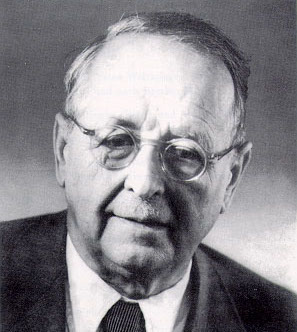August 24, 2015: what is the best Weyl asymptotic analog? One could look for a graph with n vertices and maximal eigenvalue lambda(n), one could look at the Weyl volume
```V = n/lambda(n)(d/2)
```
of the graph. The Weyl volume of a complete graph or wheel graph is 1. The Weyl Volume of the octahedron is 1, of the icosahedron 1.65836. The Weyl volume of a cyclic graph with even n is n/2. For a discrete flat torus of size nxn it is (n/3)2 if n is divisible by 3. For a 3 torus of size n, it grows like n3. In general, for triangulations with a bound on the degree, the growth is obviously the same than the number of vertices. For Barycentric refinements, the Volume goes to infinity like (d+1)m.
• August 15, 2015: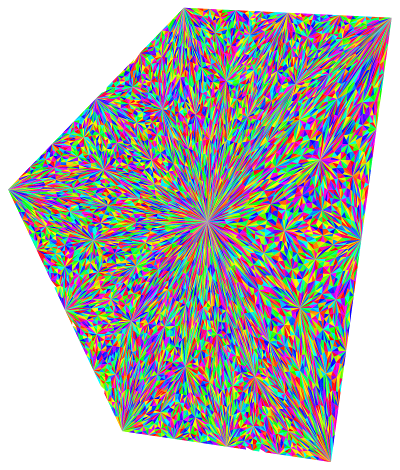Just programmed Barycentric refinement also in Euclidean setting (which does not need graph theory and is the usual setting. See picture. We defined the Barycentric refinement for any finite simple graph G as follows:
The vertices of the refinement are the complete subgraphs of G.
The edges are the pairs (a,b) of complete subgraphs, where one is contained in the other.
This definition seems not have been appeared in graph theory. I myself got to it as it is a special case of a graph product. The product of the graph with the one point graph K1.
• August 8, 2015: Mathematica code for universality. The code for the product is also in the Kuenneth paper. We have added a dimension flag "TopDim" so that the search for subsimplices is faster.
```(* Mathematica code for product  http://arxiv.org/abs/1505.07518 *)
(* Oliver Knill, June 2015                                       *)

Cl[s_,k_]:=Module[{n,t,m,u,q,V=VertexList[s],W=EdgeList[s],l},
n=Length[V]; m=Length[W]; u=Subsets[V,{k,k}]; q=Length[u]; l={};
W=Table[{W[[j,1]],W[[j,2]]},{j,m}];If[k==1,l=Table[{V[[j]]},{j,n}],
If[k==2,l=W,Do[t=Subgraph[s,u[[j]]]; If[Length[EdgeList[t]]==
Binomial[k,2],l=Append[l,VertexList[t]]], {j,q}]]];l];

Ring[s_,a_]:=Module[{v,n,m,u,X},v=VertexList[s]; n=Length[v];
u=Table[Cl[s,k],{k,TopDim+1}] /. Table[k->a[[k]],{k,n}];m=Length[u];
X=Sum[Sum[Product[u[[k,l,m]],
{m,Length[u[[k,l]]]}],{l,Length[u[[k]]]}],{k,m}]];

GR[f_]:=Module[{s={}},Do[Do[If[Denominator[f[[k]]/f[[l]]]==1 && k!=l,
s=Append[s,k->l]],{k,Length[f]}],{l,Length[f]}];
UndirectedGraph[Graph[s]]];

GraphProduct[s1_,s2_]:=Module[{f,g,i,fc,tc},
fc=FromCharacterCode; tc=ToCharacterCode;
i[l_,n_]:=Table[fc[Join[tc[l],IntegerDigits[k]+48]],{k,n}];
f=Ring[s1,i["a",Length[VertexList[s1]]]];
g=Ring[s2,i["b",Length[VertexList[s2]]]]; GR[Expand[f*g]]];

NewGraph[s_]:=GraphProduct[s,CompleteGraph];
Bary[s_,n_]:=Last[NestList[NewGraph,s,n]];

TopDim=2; GraphProduct[CompleteGraph,StarGraph] (* example *)
TopDim=3; Bary[CompleteGraph,2]                    (* example *)

(* Code for universality  http://arxiv.org/abs/1508.02027v1      *)
(* Oliver Knill, August 8 2015                                   *)

Laplace[s_,n_]:=Normal[KirchhoffMatrix[Bary[s,n]]];
Spectrum[s_,n_]:= Sort[Eigenvalues[1.0*Laplace[s,n]]];
F[s_,n_]:=ListPlot[Spectrum[s,n],Filling -> Bottom];
TopDim=1; F[StarGraph,6]     (* 1D case completely understood *)
TopDim=2; F[CompleteGraph,3] (* d=2,m=3, for m=4, wait longer *)
TopDim=3; F[CompleteGraph,2] (* d=3,m=2, for m=3, wait longer *)
```
Here is what is seen in the case d=2,m=3:• The paper was written rather quickly and thrown out pretty fast because it is very exciting for me as it ties in with topics, I had worked on as a graduate student. The renormalization of Jacobi matrices was part of my thesis. When trying to Toda deform higher dimensional Schroedinger operators, the factorization appeared to matter (PDF). Later I realized how to deform the exterior derivative in a graph or Riemannian manifold, which produces an integrable system with exciting properties. Also there the factorization of the Laplacian L=D2 was crucial. As I see it now, the two things can be decoupled and work independently if one looks at the Dirac operator D rather than the Laplacian. Its always possible to isospectrally deform the Dirac operator D (in the Riemannian case D=d+d*) is sometimes also called the Euler operator) and it is also always possible to get to selfsimilar limiting graphs in any dimension (by applying Barycentric refinements). While in dimension 1, the dynamics of the quadratic map matters (on a special Julia set), in higher dimensions, its still foggy, what underlying dynamical system is there in higher dimensions.